# GSEB Solutions Class 12 Accounts Part 1 Chapter 6 Retirement / Death of a Partner

Gujarat Board GSEB Textbook Solutions Class 12 Commerce Accounts Part 1 Chapter 6 Retirement / Death of a Partner Textbook Exercise Questions and Answers.

## Gujarat Board Textbook Solutions Class 12 Accounts Part 1 Chapter 6 Retirement / Death of a Partner

### GSEB Class 12 Accounts Retirement / Death of a Partner Text Book Questions and Answers

Question 1.
Select appropriate alternative for each question :

(1) Debit balance of profit and loss account shown in the balance sheet at the time of retirement
– of a partner is ……………..
(A) recorded on the debit side of all partners’ capital accounts including the retiring partner in their old profit-loss sharing ratio.
(B) recorded on the credit side of all partners’ capital accounts including the retiring partner in their old profit-loss sharing ratio.
(C) credit side of the retiring partners’ capital account only.
(D) debit side of the remaining partners’ capital accounts in their gaining ratio.
(A) recorded on the debit side of all partners’ capital accounts including the retiring partner in their old profit-loss sharing ratio.(2) Goodwill shown in the balance sheet at the time of the retirement of a partner is recorded
as ……………….
(A) shown in new balance sheet, if decided by partners.
(B) debit side of all partners’ capital accounts in their old profit-loss sharing ratio.
(C) credit side of all partners’ capital accounts in their old profit-loss sharing ratio.
(D) debit side of retiring partners’ capital account only.
(B) debit side of all partners’ capital accounts in their old profit-loss sharing ratio.

(3) Goodwill payable to the retiring partner is recorded as ………………..
(A) credit side of all partners’ capital accounts, in their old profit-loss sharing ratio.
(B) credit side of all partners’ capital accounts, in their gaining ratio.
(C) debit side of continuing partners’ capital accounts, in their gaining ratio.
(D) debit side of continuing partners’ capital accounts, in their new profit-loss sharing ratio.
(C) debit side of continuing partners’ capital accounts, in their gaining ratio.

(4) When only old profit-loss sharing ratio is given, gaining ratio of remaining partners will be
(A) 1 : 1
(B) old ratio
(C) capital ratio
(D) can not be calculated
(B) old ratio

(5) A partner, except the retiring partner also receives goodwill when ……………….
(A) his capital is more.
(B) new share in new profit-loss sharing ratio is more than his old share.
(C) new share in new profit-loss sharing ratio is less than his old share.
(D) new share and old share are equal.
(C) new share in new profit-loss sharing ratio is less than his old share.

(6) Loss of revaluation account at the time of retirement or death is recorded in ……………… account in ………………… ratio on ……………….. side of the capital accounts.
(A) remaining partners, new profit-loss sharing, debit
(B) all partners, old profit-loss sharing, credit
(C) all partners, old profit-loss sharing, debit
(D) all partners, equal proportion, debit
(C) all partners, old profit-loss sharing, debit

(7) If partnership deed is silent, interest is payable at ……………….. on unpaid amount payable to the retiring partner.
(A) 10 % p.a.
(B) 12 % p.a.
(C) 6 % p.a.
(D) zero
(C) 6 % p.a.(8) Accounting year ends on 31-3-2016. A partner dies on 30-6-2016. Deceased partners’ share in profit is $$\frac {1}{3}$$ . Profit share payable to the partner is to be calculated on the basis of last year’s profit ₹ 24,000 …………….. amount will be paid as share in profit at the time of death.
(A) ₹ 8,000
(B) ₹ 24,000
(C) ₹ 1,333
(D) ₹ 2,000
(D) ₹ 2,000

(9) Sweta, Geeta and Jyoti are equal partners. Gita retires. Gita’s share is gained by Sweta and Jyoti equally. New profit and loss sharing ratio of Sweta and Jyoti will be ………………
(A) 3 : 1
(B) 2 : 1
(C) 1 : 2
(D) 1 : 1
(D) 1 : 1

(10) Workmen profit sharing fund is recorded as at the time of the retirement of a partner.
(A) a liability in new balance sheet.
(B) credited to all partners’ capital account in their old profit-loss sharing ratio.
(C) debited to all partners’ capital account in their old profit-loss sharing ratio.
(D) credited to the retiring partners’ capital account.
(A) a liability in new balance sheet.

Question 2.
Answer the following questions in one sentence :

(1) State the circumstances of the retirement of a partner.
The circumstances of the retirement of a partner are as follows :

• As per Indian Partnership Act.
• Voluntary retirement of a partner due to his personal reason.

(2) Which important accounting aspects are considered at the time of the retirement or death of a partner ?
Following important accounting aspects are considered at the time of the retirement or death of a partner :

• Calculation of new profit-loss sharing ratio and calculation of gaining share of continuing partners,
• Valuation of goodwill and its accounting treatment,
• Revaluation of assets and Liabilities,
• Distribution of accumulated reserves and balances of undistributed profit and loss,
• Determination of amount payable to the retiring or deceased partner,
• Payment of amount due to the retiring or deceased partner.

(3) Which balances are credited to all partners’ capital accounts in their old profit-loss sharing ratio ?
At the time of retirement of death of a partner, undistributed balances of profit and accumulated reserves shown in the balance sheet are credited to all the partners’ capital/current account in old profit sharing ratio.(4) Which balances are debited to all partners’ capital accounts in their old profit-loss sharing ratio ?
At the time of retirement or death of a partner, balance of undistributed losses and deferred revenue expenditure shown in the balance sheet are debited to all the partners’ capital/current account in old profit sharing ratio.

(5) Explain accounting treatment of old goodwill appearing in the balance sheet of the firm at the time of the retirement of a partner.
At the time of the retirement of a partner, goodwill appear in the balance sheet of a firm distributed among partners in their old profit- loss ratio. Journal entry for that.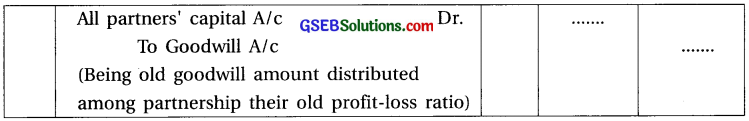(6) Explain accounting treatment of the new goodwill of the firm valued at the time of the retirement of a partner.
At the time of retirement or death of a partner, retiring or deceased partner is given his share of goodwill to continuing partners in their gaining ratio. Entry will be as under :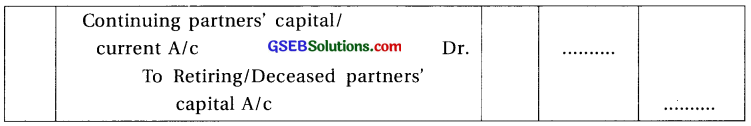(7) When and why the profit and loss adjustment account (Revaluation Account) is prepared ? Answer:
At the time of the retirement or death of a partner to record the accounting effects of
revaluation of assets and liabilities, profit and loss adjustment account is preapared.

(8) Who gives the share in goodwill to the retiring or deceased partner ? Why ?
Because of retirement or death of a partner, continuing partners will get his share of profit and goodwill will be given to retiring or deceased partner of his share.Question 3.
(1) X, Y and Z are the partners sharing profit and loss in the ratio of 5 : 3 : 2, Z retires.
Old ratio of partners X, Y and Z = 5 : 3 : 2. Partner Z retires and no clarification regarding new ratio. So the new ratio of X and Y will be their old ratio = 5 : 3. Further gain ratio also will be old profit and loss ratio i.e. 5 : 3.

(2) A, B and C are the partners sharing profit and loss in the ratio of $$\frac {1}{2}$$,$$\frac {1}{3}$$, and $$\frac {1}{6}$$ respectively.
(A) If A retires (B) If B retires (C) If C retires.
Old profit and loss ratio of partners A, B and C ⇒ 3 : 2 : 1.
Now, here no other information related to the new profit-loss ratio is given, old ratio of continuing partners remains as new profit-loss ratio and gaining ratio.
(i) If A retires ⇒ New profit-loss ratio of partner B and C = 2 : 1 ; New gaining ratio = 2 : 1
(ii) If B retires ⇒ New profit-loss ratio of partner A and C = 3 : 1 ; New gaining ratio = 3 : 1
(iii) If C retires ⇒ New profit-loss ratio of partner A and B = 3 : 2 ; New gaining ratio = 3 : 2

(3) P, Q and R are the partners sharing profit and loss in the ratio of 40%, 20% and 40%
respectively. Q retires. P is acquiring $$\frac {3}{20}$$ and R is acquiring $$\frac {1}{20}$$ from Q’s share.
Old ratio of P, Q and R = ⇒ $$\frac{40}{100}: \frac{20}{100}: \frac{40}{100}$$ = 4 : 2 : 4 = 2 : 1 : 2
Q is retires and P acquires $$\frac {3}{20}$$ th share and R acquires $$\frac {1}{20}$$th share of Q.

∴ Gain of P = $$\frac {3}{20}$$, Gain of R = $$\frac {1}{20}$$ ∴ Gaining ratio of P and R = 3 : 1
New share = Old share + Gain
P’s new share = $$\frac{2}{5}+\frac{3}{20}=\frac{8+3}{20}=\frac{11}{20}$$; R’s new share = $$\frac{2}{5}+\frac{1}{20}=\frac{8+1}{20}=\frac{9}{20}$$
∴ New profit and loss sharing ratio of P and R = 11 : 9

(4) M, N and O are the partners sharing profit and loss in the ratio of 3 : 2 : 1. M retires, N is acquiring $$\frac {1}{10}$$ from M’s share and balance is acquired by O.
Old profit-loss ratio of M, N and O = 3 : 2 : 1.
M retires. N receives $$\frac {1}{10}$$ from M’s share.
∴ O’s gain = M’s share -N’s gain = $$\frac{3}{6}-\frac{1}{10}=\frac{15-3}{30}=\frac{12}{30}$$
N receives $$\frac{1}{10}=\frac{3}{30}$$ and Q receives = $$\frac {12}{30}$$ .
∴ Gaining ratio for N and O = $$\frac{3}{30}: \frac{12}{30}$$ = 3 : 12 = 1 : 4
New share = Old share + Gain
∴ N’s new Share = $$\frac{2}{60}+\frac{3}{30}=\frac{10+3}{30}=\frac{13}{30}$$ ; O’s new share = $$\frac{1}{6}+\frac{12}{30}=\frac{5+12}{30}=\frac{17}{30}$$
∴ New profit and loss sharing ratio for N and O = 13 : 17

(5) C, B and D are the partners sharing profit and loss in the ratio of 4 : 5 : 3. D retires and his share is taken up by C and B in the ratio of 2 : 1.
Old profit-loss ratio of partners C, B and D = 4 : 5 : 3
Partners D retires. Profit share of D is acquired by C and B in 2 : 1 ratio.
∴ Gaining share of continuing partner = Share of the retiring partner × Gaining share
given to the resp. partners
∴C’s gain = $$\frac{3}{12} \times \frac{2}{3}=\frac{6}{36}$$ ; B’s gain = $$\frac{3}{12} \times \frac{1}{3}=\frac{3}{36}$$
∴ Gaining ratio for C and B = 6 : 3 = 2 : 1
Now, New share = Old share + Gain
∴ C’s new share = $$\frac{4}{12}+\frac{6}{36}=\frac{12+6}{36}=\frac{18}{36}=\frac{1}{2}$$ ; B’s new share = $$\frac{5}{12}+\frac{3}{36}=\frac{15+3}{36}=\frac{18}{36}=\frac{1}{2}$$
∴ New profit-loss sharing ratio for partners C and B = 1 : 1(6) A, M and C are the partners sharing profit and loss in the ratio of 3 : 5 : 2. M retires and his share is taken up by C only.
Old profit-loss sharing ratio of A, M and C = 3 : 5 : 2.
M retires and his $$\frac {5}{10}$$ share is taken by C only. ∴ C’s gain = $$\frac {5}{10}$$ and A’s gain = 0
New share = Old share + Gain
A’s New share = $$\frac {3}{10}$$ + 0 = $$\frac {3}{10}$$ ; C’s new share = $$\frac{2}{10}+\frac{5}{10}=\frac{2+5}{10}=\frac{7}{10}$$
∴ New profit-loss sharing ratio for Aand C = 3 : 7

(7) P, Q, R and S are the partners sharing profit and loss in the ratio of 4 : 3 : 2 : 1. Q retires and his share of profit is gained equally by R and S.
Q retires and his share is gained by R and S equally i.e. 1 : 1.
Now, Gain = share of retiring partner × gain share
∴ R’s gain = $$\frac{3}{10} \times \frac{1}{2}=\frac{3}{20}$$ ; S’s gain = $$\frac{3}{10} \times \frac{1}{2}=\frac{3}{20}$$
Now, new share = old share + gain
∴ P’s new share = $$\frac{4}{10}$$ + 0 = $$\frac{4}{10}$$ ; R’s new share = $$\frac{2}{10}+\frac{3}{20}=\frac{4+3}{20}=\frac{7}{20}$$
S’s new share = $$\frac{1}{10}+\frac{3}{20}=\frac{2+3}{20}=\frac{5}{20}$$
∴ New profit-loss sharing ratio of P, R and S = $$\frac{4}{10}: \frac{7}{20}: \frac{5}{20}$$ = 8 : 7 : 5

(8) M, N, O and P are the partners sharing profit and loss in the ratio of 5 : 3 : 2 : 2. N and P retired. N’s share is acquired by O and P’s share is acquired by M.
Old ratio of M, N, O and P = $$\frac{5}{12}: \frac{3}{12}: \frac{2}{12}: \frac{2}{12}$$. Here, N and P retired.
$$\frac{2}{12}$$ share of P is acquired by M and $$\frac{3}{12}$$ share of N is acquired by O.
∴ Gain of M = $$\frac{2}{12}$$ and gain of O = $$\frac{3}{12}$$
∴ Gain ratio of M and 0 = 2 : 3
Now, New share = Old share + Gain
New share of M = $$\frac{5}{12}+\frac{2}{12}=\frac{7}{12}$$
New share of O = $$\frac{2}{12}+\frac{3}{12}=\frac{5}{12}$$
New profit – loss ratio M and 0 = 7 : 5

(9) A, B and C are the partners sharing profit and loss in the ratio of $$\frac {1}{2}$$, 30% and $$\frac {1}{5}$$ respectively. B’s share is taken over by A and C in the ratio of 3 : 2.
Profit and loss sharing ratio of partners A, B and C = $$\frac{1}{2}: \frac{30}{100}: \frac{1}{5}=\frac{1}{2}: \frac{3}{10}: \frac{1}{5}$$ = 5 : 3 : 2
Partners A and C receives benefit in 3 : 2 ratio ∴ Gaining ratio for A and C = 3 : 2.
Gain = share of retiring partner x gain share
A’s gain = $$\frac{3}{10} \times \frac{3}{5}=\frac{9}{50}$$ ; C’s gain = $$\frac{3}{10} \times \frac{2}{5}=\frac{6}{50}$$
New share = Old share + Gain
A’s new share = $$\frac{5}{10}+\frac{9}{50}=\frac{25+9}{50}=\frac{34}{50}$$ ; C’s new share = $$\frac{2}{10}+\frac{6}{50}=\frac{10+6}{50}=\frac{16}{50}$$
New profit – loss ratio of A and C = $$\frac{34}{50}: \frac{16}{50}$$ = 17 : 8(10) A, B and C are the partners sharing profit and loss in the ratio of 5 : 3 : 2. B and C sharing profit in the ratio of 40% and 60% after the retirement of A.
After retirement of A, New ratio for B and C = 2 : 3
Now, Gain = New share – Old share
B’s gain = $$\frac{2}{5}-\frac{3}{10}=\frac{4-3}{10}=\frac{1}{10}$$ ; C’s gain = $$\frac{3}{5}-\frac{2}{10}=\frac{6-2}{10}=\frac{4}{10}$$
∴ Gaining ratio for partners B and C = 1 : 4 ; New profit-loss ratio for B and C = 2 : 3

(11) A, B and C are the partners sharing profit and loss in the ratio of 3 : 2 : 1. C retires. The new profit and loss sharing ratio of A and B is decided at 7 : 5.
Old profit and loss ratio for A, B and C = 3 : 2 : 1
After retirement of C, new ratio for A and B = 7 : 5
Now, Gain = New share – Old share
A’s gain = $$\frac{7}{12}-\frac{3}{6}=\frac{7-6}{12}=\frac{1}{12}$$ ; B’s gain = $$\frac{5}{12}-\frac{2}{6}=\frac{5-4}{12}=\frac{1}{12}$$
∴ Gaining ratio for A and B = 1 : 1 ; New profit-loss ratio for A and B = 7 : 5

(12) A, B and C are the partners sharing profit in the ratio of 4 : 5 : 1. Following journal entry for goodwill is passed at the time of the retirement of B.
A’s capital A/c Dr 6,000
C’s capital A/c Dr 4,000
To B’s capital A/c 10,000
From the capital amount of A and C, we can say that gaining ratio for A and C = 3 : 2.
Now, Gain = Share of retiring partner × Gain Share
A’s gain = $$\frac{5}{10} \times \frac{3}{5}=\frac{15}{50}$$ ; C’s gain = $$\frac{5}{10} \times \frac{2}{5}=\frac{10}{50}$$ ∴ Gaining ratio = $$\frac{15}{50}: \frac{10}{50}$$ = 3 : 2
Now, New share = Old share + Gain
A’s ne wshare= $$\frac{4}{10}+\frac{15}{50}=\frac{20+15}{50}=\frac{35}{50}$$ ; C’s new share = $$\frac{1}{10}+\frac{10}{50}=\frac{5+10}{50}=\frac{15}{50}$$
∴ New profit-loss sharing ratio for A and C = $$\frac{35}{50}: \frac{15}{50}$$ = 7 : 3

(13) A, B, C and D are the partners sharing profit and loss in the ratio of 4 : 3 : 2 : 1. C retires. After the retirement of C, A will maintain his old profit share.
C retires, at that time A will maintain his old profit share ∴ Gaining ratio for B and D = 3 : 1. Gain = Share of retiring partner × Gain share
B’s gain = $$\frac{2}{10} \times \frac{3}{4}=\frac{6}{40}$$ ; D’s gain = $$\frac{2}{10} \times \frac{1}{4}=\frac{2}{40}$$
New share = Old share + Gain
A’s new share = $$\frac{4}{10}$$ + 0 = $$\frac{4}{10}$$ ;B’s new share = $$\frac{3}{10}+\frac{6}{40}=\frac{12+6}{40}=\frac{18}{40}$$;
D’s new share = $$\frac{1}{10}+\frac{2}{40}=\frac{4+2}{40}=\frac{6}{40}$$
∴ New profit-loss sharing ratio for partners
A, B and D = $$\frac{4}{10}: \frac{18}{40}: \frac{6}{40}$$ = 16 : 18 : 6 = 8 : 9 : 3

Question 4.
Give necessary entry of goodwill for the following :
(1) Akruti, Prakruti and Sanskruti are the partners sharing profit and loss in the ratio of 5 : 3 : 2. Sanskruti retires. At the time of her retirement the goodwill is valued at ₹ 30,000.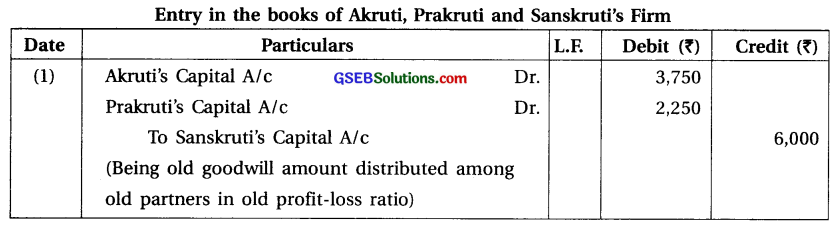(2) X, Y and Z are the partners sharing profit and loss in equal proportions. Goodwill appears at ₹ 42,000 in the books of the firm. At the time of retirement of X, the goodwill of the firm is valued at ₹ 1,20,000.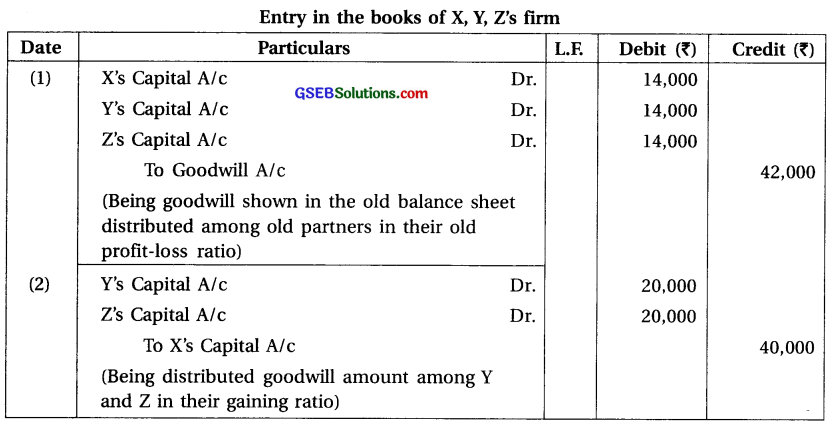Note :
(1) Here, new profit – loss ratio or gaining ratio is not given so old ratio of Y and
Z = 1 : 1 will be the new profit – loss ratio and gaining ratio.
(2) Share of goodwill of X = ₹ 1,20,000 × $$\frac {1}{3}$$ = ₹ 40,000 which will be given to Y and Z in gain ratio of 1 : 1.
(3) Amount of old goodwill shown in the balance sheet will be written off between all partners in the old ratio (1 : 1 : 1).

(3) L, M, N and O are the partners sharing profit and loss in the ratio of 5 : 4 : 3 : 3. L retires on 1-4-2017. At the time of retirement of L, goodwill appears at ₹ 75,000 in the books of old firm. The new profit and loss sharing ratio of M, N and O is decided at 3 : 1 : 1. On L’s retirement, the goodwill of the firm is valued at ₹ 90,000.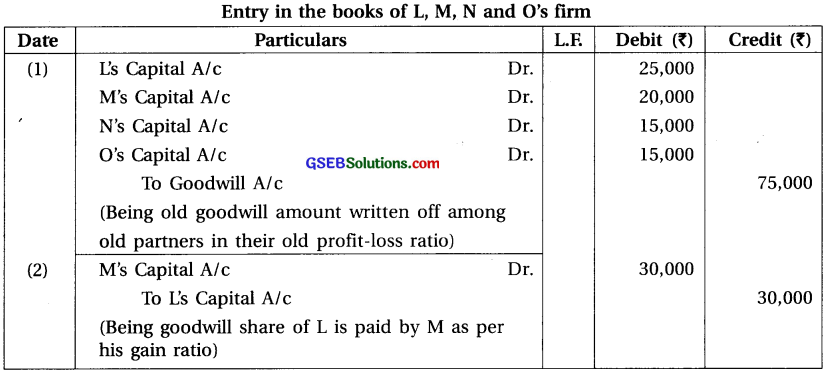Note :
(1) Gaining Ratio :
Old ratio of L, M, N and O = 5 : 4 : 3 : 3 and New ratio of M, N and O = 3 : 1 : 1.
∴ Gain = New share – Old share
∴ Gain of M = $$\frac{3}{5}-\frac{4}{5}=\frac{9-4}{15}=\frac{5}{15}$$
∴ Gain of N = $$\frac{1}{5}-\frac{3}{5}=\frac{3-3}{15}$$ = 0
∴ Gain of O = $$\frac{1}{5}-\frac{3}{5}=\frac{3-3}{15}$$ = 0
∴ Here, only M gets gain, so goodwill share of L will be given to only M.

(2) Goodwill share of L = ₹ 90,000 × $$\frac {5}{15}$$ = ₹ 30,000

(3) Amount of old goodwill shown in the balance sheet will be written off between all partners in old ratio (5 : 4 : 3 : 3).

(4) A, B and C are the partners of a firm. B retires. At the time of B’s retirement, the goodwill of
the firm is valued at ₹ 60,000. The new profit-loss sharing ratio of A and C decided at 7 : 2.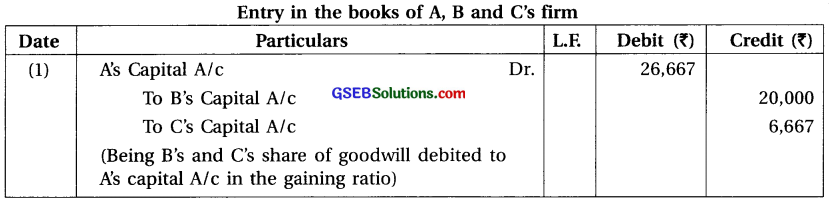Note : (1) Gaining Ratio :
Old ratio of A, B and C = 1 : 1 : 1. New ratio of A and C = 7 : 2
Gain = New share – Old share
∴ Gain of A = $$\frac{7}{9}-\frac{1}{3}=\frac{7-3}{9}=\frac{4}{9}$$
∴ Gain of C = $$\frac{2}{9}-\frac{1}{3}=\frac{2-3}{9}=\frac{-1}{9}$$ (sacrifice)

(2) Revalued goodwill amount = ₹ 60,000. A, B and C are partners sharing profit-loss equally.
∴ Goodwill receivable by B = ₹ 20,000. Now this goodwill amount distributed . among partners m new ratio = $$\frac{60,000}{9}$$ = ₹ 6,667.(5) B, R, T and S are the partners sharing profit and loss in the ratio of 4 : 3 : 1 : 2. B retires. Goodwill is appearing in their books at ₹ 20,000 and at the time of B’s retirement, goodwill is valued at ₹ 60,000. R, T and S decided to share the future profits of new firm in the ratio of 1 : 2 : 2.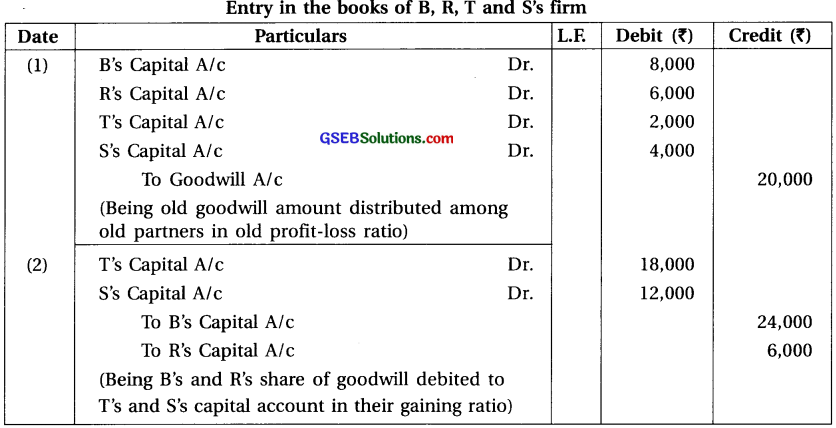Note :
(1) Gaining Ratio :
Old ratio of B, R, T and S = 4 : 3 : 1 : 2. New ratio of R, T and S = 1 : 2 : 2.
Gain = New share – Old share
∴ Gain of R = $$\frac{1}{5}-\frac{3}{10}=\frac{2-3}{10}=\frac{-1}{10}$$ (Sacrifice)
∴ Gain of T = $$\frac{2}{5}-\frac{1}{10}=\frac{4-1}{10}=\frac{3}{10}$$
∴ Gain of S = $$\frac{2}{5}-\frac{2}{10}=\frac{4-2}{10}=\frac{2}{10}$$
Gaining ratio of Tand S = 3 : 2

(2) Amount of goodwill received by retired partner B = ₹ 6,00,000 × $$\frac{4}{10}$$ = ₹ 24,000 Because of R sacrifice his share, so amount of goodwill received by
R = ₹ 60,000 × $$\frac{1}{10}$$ = ₹ 6,000.
Total amount of goodwill ₹ 24,000 + ₹ 6,000 = ₹ 30,000.
This amount will be debited to Capital Account of T and S in their gaining ratio (3 : 2).

(6) A, M, U and L are the partners sharing profit and loss in the ratio of 6 : 4 : 3 : 2. U retires. His capital account after making adjustments for reserves and profit on revaluation is ? 80,000. Remaining partners have agreed to pay him ? 1,40,000 in full settlement of his claim. The new profit-loss sharing ratio of A, M and L is decided at 6 : 5 : 4 after the retirement of U.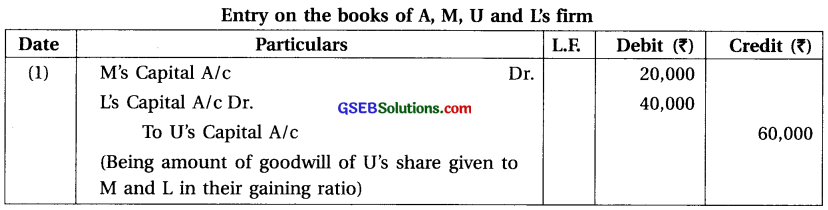Note :
(1) Gaining Ratio :
Old ratio of A, M, U and L = 6 : 4 : 3 : 2. New ratio of A, M and L = 6 : 5 : 4.
Gain = New share – Old share
∴ Gain of A = $$\frac{6}{15}-\frac{6}{15}=\frac{6-6}{15}$$ = 0
∴ Gain of M = $$\frac{5}{15}-\frac{4}{15}=\frac{5-4}{15}=\frac{1}{15}$$
∴ Gain of L = $$\frac{4}{15}-\frac{2}{15}=\frac{4-2}{15}=\frac{2}{15}$$
∴ Gaining ratio of M and L = 1 : 2.

(2) Amount of Goodwill: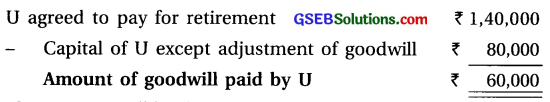This amount will be debited to capital account of M and L is their gaining ratio (1: 2).Question 5.
Dhaval, Kamal and Naval are the partners sharing profit and loss in the ratio of 2 : 2 : 1. Naval retires on 31-3-2016. Balance sheet of the firm as on 31-3-2016 was as under :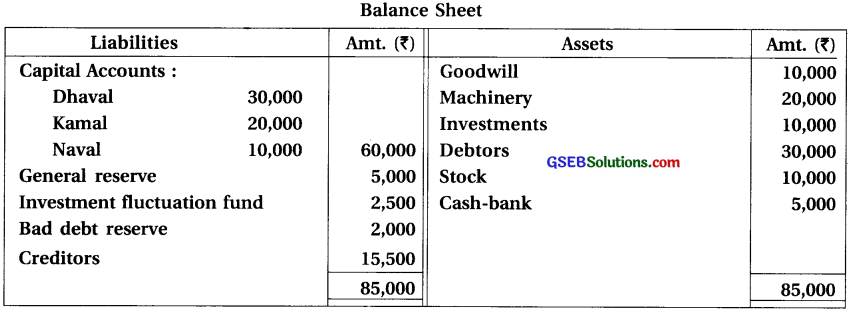Following adjustments are agreed at the time of retirement :
(1) Value of machinery is ₹ 25,000 and Value of stock is ₹ 5,000. (2) Value of investments is ₹ 8,000, which is taken by Naval at this price. (3) An amount of ₹ 5,000 included in creditors is no longer payable. (4) The provision for workmen compensation to be credited at ₹ 2,000. (5) The provision for doubtful debts is to be kept at 10% on debtors. (6) Goodwill of the firm is valued at ₹ 40,000.
Pass journal entries. Prepare necessary accounts and the balance sheet of the firm after Naval’s retirement.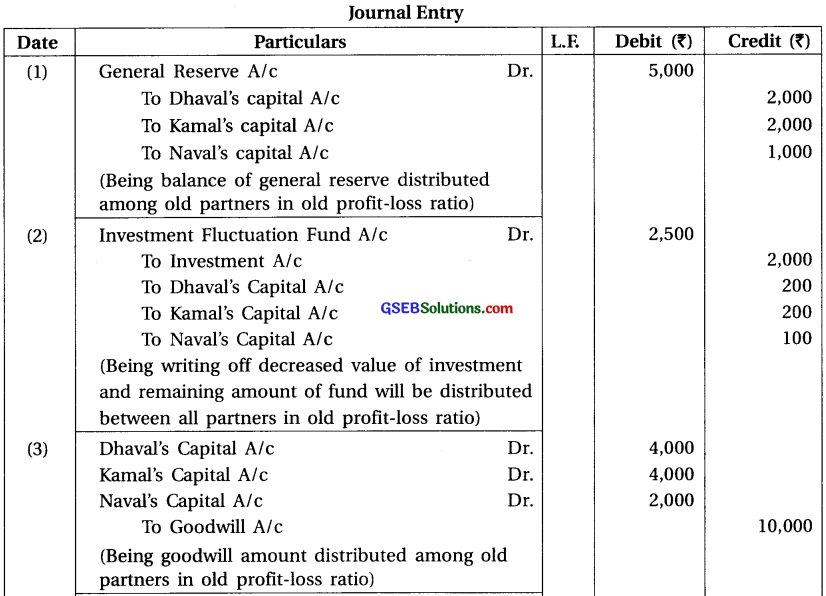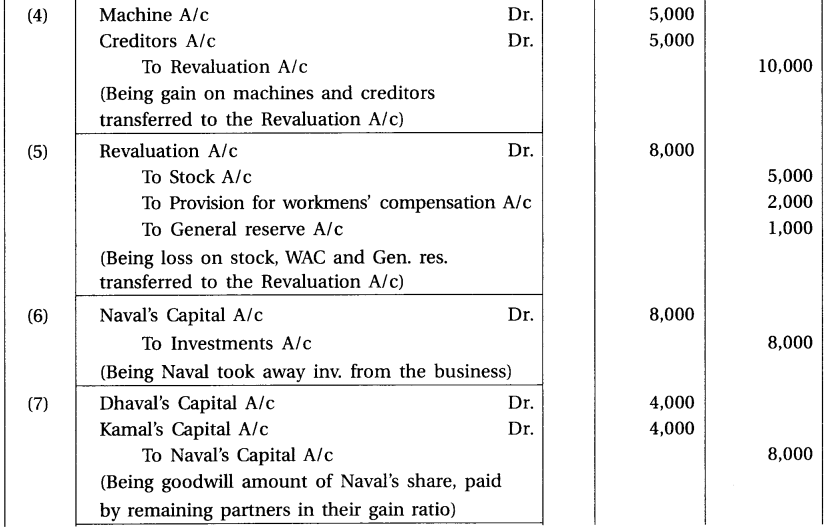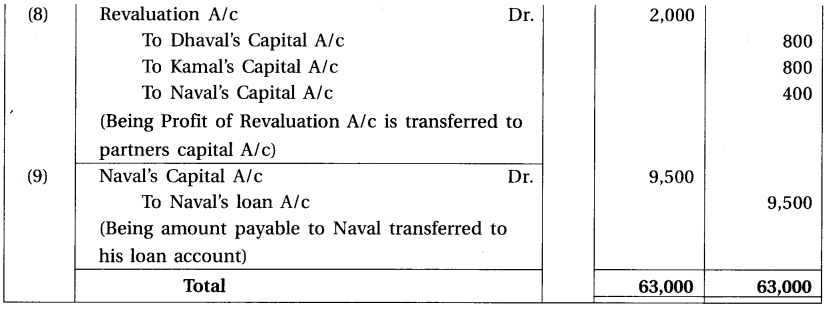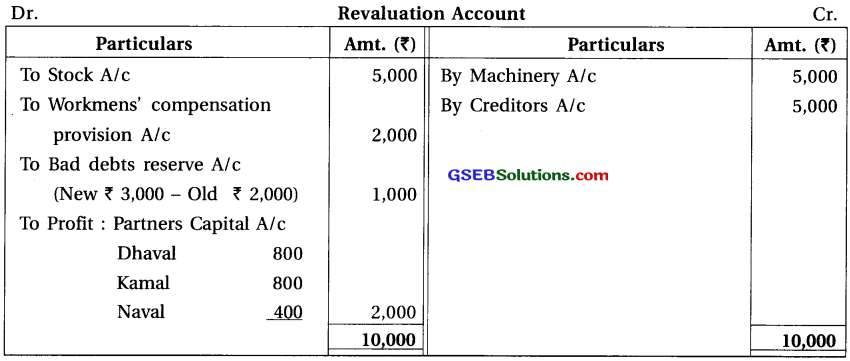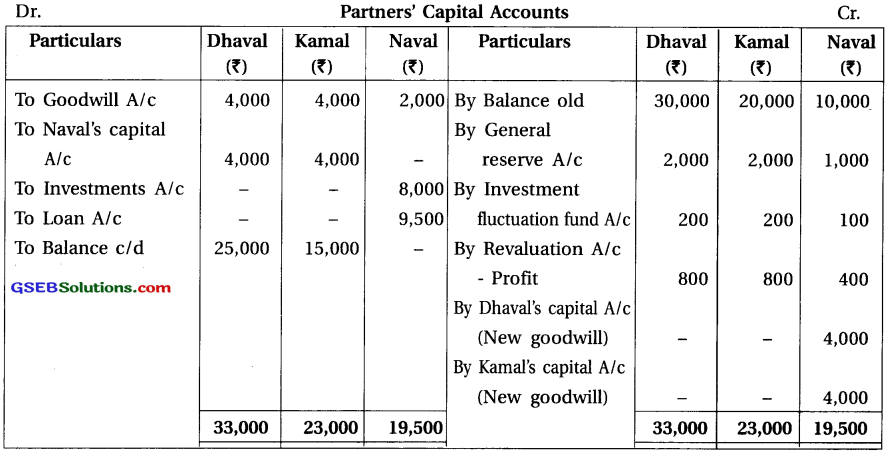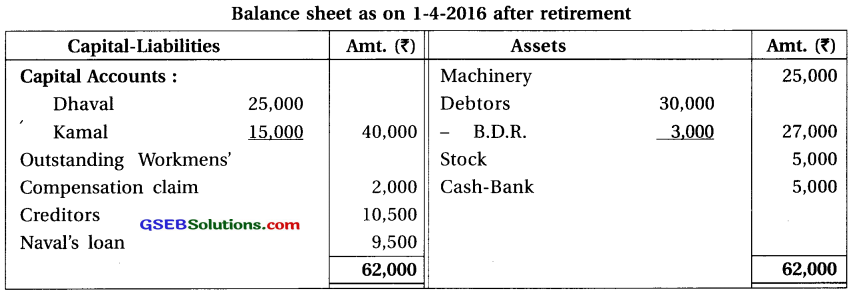Note :
(1) Old ratio of Dhaval, Kamal and Naval = 2 : 2 : 1. New ratio after retirement of Naval
is not given ∴ New ratio of Dhaval and Kamal = Old ratio = Gaining ratio = 2 : 2 = 1 : 1.

(2) Amount of old goodwill shown in the balance sheet ₹ 10,000 will be distributed between all partners in old ratio (2 : 2 : 1).

(3) Goodwill share of Naval = 40,000 × $$\frac {1}{5}$$ = 8,000 which will be given to Dhaval and Kamal in their gaining ratio (1 : 1).Question 6.
Rohit, Mohit and Virat are partners sharing profit and loss in the ratio of 4 : 3 : 2. Balance sheet of the firm as on 31-3-2017 was as under :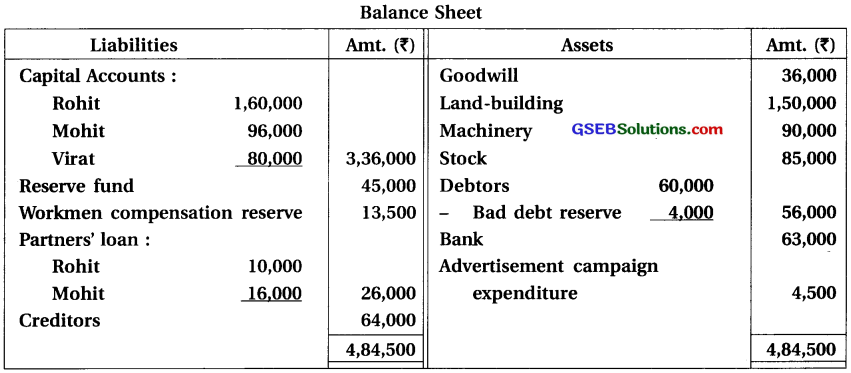Rohit retired on 1-4-2017. Terms of retirement is as under :
(1) Value of land-building is ₹ 1,80,000. (2) Value of machinery is to be reduced by ₹ 15,000.
(3) Provision for doubtful debts is to be kept at 10% on debtors. (4) ₹ 5,000 not payable to creditors. (5) Valuation of goodwill is ₹ 1,80,000. (6) New profit-loss sharing ratio of Mohit and Virat is 2 : 1. (7) ₹ 20,000 are to be paid to Rohit and balance will be kept as loan.
Prepare Revaluation account, Partners’ capital accounts and Balance sheet after retirement.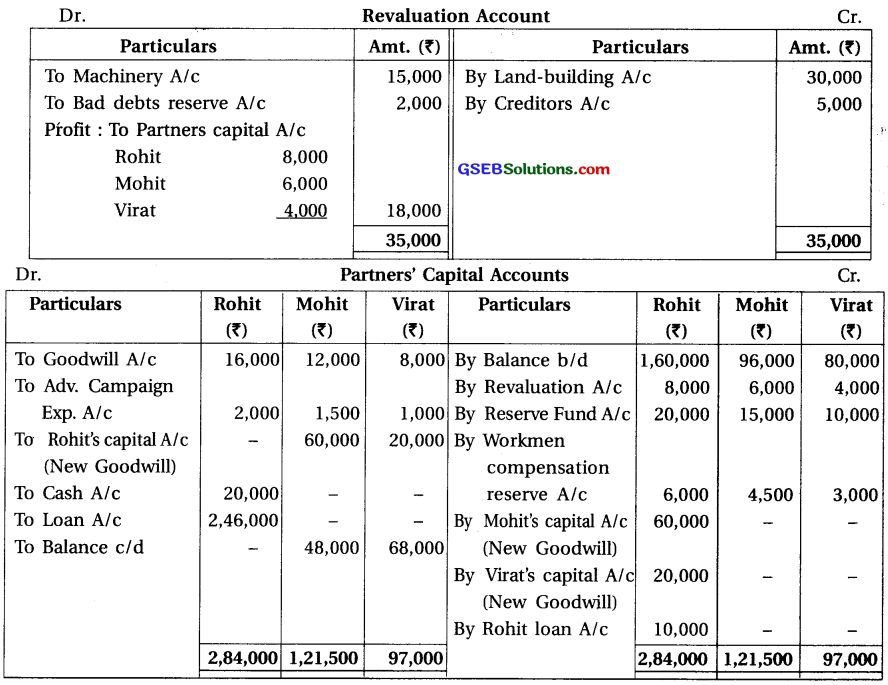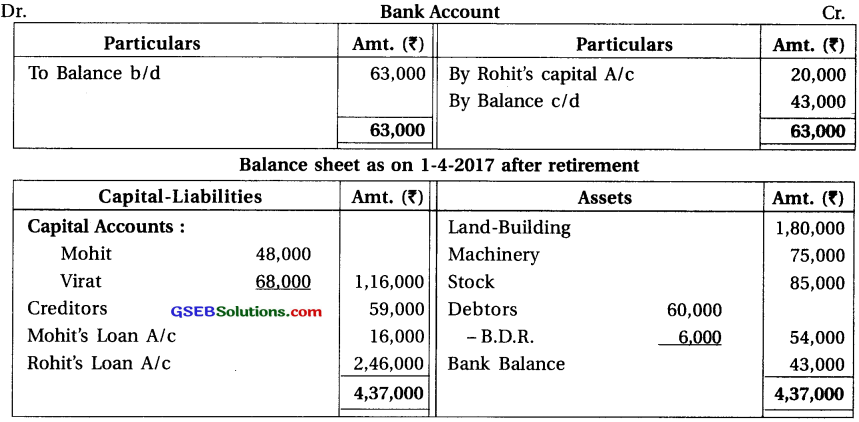Note :
(1) Gaining Ratio : Old ratio of Rohit, Mohit and Virat = 4 : 3 : 2.
New ratio of Mohit and Virat = 2 : 1.
Gain = New share – Old share
Gain of Mohit = $$\frac{2}{3}-\frac{3}{9}=\frac{6-3}{9}=\frac{3}{9}$$
Gain of Virat = $$\frac{1}{3}-\frac{2}{9}=\frac{3-2}{9}=\frac{1}{9}$$
Gaining ratio of Mohit and Virat = 3 : 1.

(2) Share in goodwill of Rohit = ₹ 1,80,000 × $$\frac{4}{9}$$ = ₹ 80,000 which will be given to Mohit and Virat in their gaining ratio (3 : 1)

(3) Amount of old goodwill shown in the balance sheet ₹ 36,000 will be distributed between old partner in their old ratio (4 : 3 : 2).Question 7.
Vijay, Laxmi and Siddhi are the partners sharing profit and loss in the ratio of 5 : 3 : 2. Siddhi retired on 1-4-2016. Terms of retirement are as under :

(1) New profit-loss sharing ratio of Vijay and Laxmi is 2 : 3. (2) Goodwill of the firm is valued at ₹ 60,000. (3) Market value of investments is ₹ 40,000. Siddhi will take over investment at this value. (4) ₹ 3,000 to be written off from debtors and 5% bad debt reserve is to be maintained. (5) Value of stock shown in the book is ₹ 1,000 more than its cost. It is to be recorded at cost. (6) Claim of ₹ 7,000 is accepted for workmen compensation. (7) ₹ 12,000 to be paid to Siddhi immediately.
Balance sheet of the firm on 31-3-2016 was as under :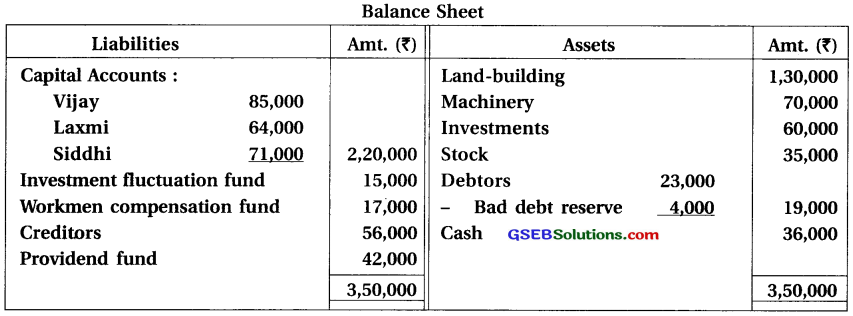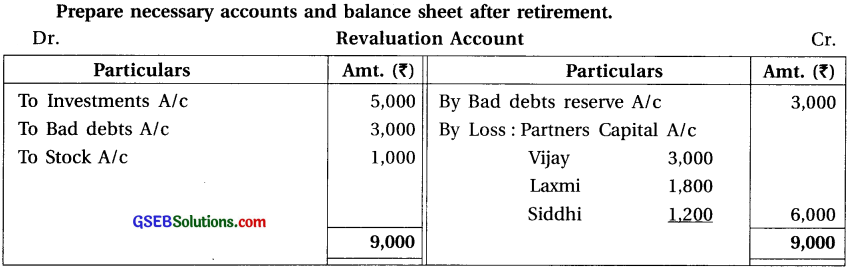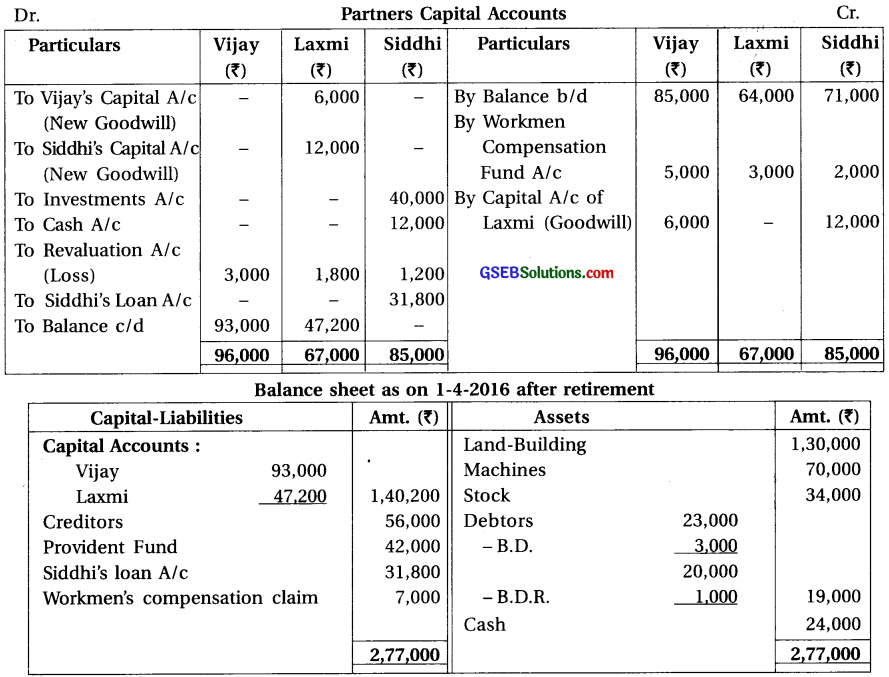Note :
(1) Gaining Ratio : Old ratio of Vijay, Laxmi and Siddhi = 5 : 3 : 2.
New ratio of Vijay and Laxmi = 2 : 3. Gain = New share – Old share
∴ Gain of Vijay = $$\frac{2}{5}-\frac{5}{10}=\frac{4-5}{10}=\frac{-1}{10}$$ (sacrifice)
∴ Gain of Laxmi = $$\frac{3}{5}-\frac{3}{10}=\frac{6-3}{10}=\frac{3}{10}$$ ∴ Here only Laxmi has gain.

(2) Goodwill :
Goodwill received by Siddhi = ₹ 60,000 × $$\frac{2}{10}$$ = ₹ 12,000
Goodwill received by Vijay = ₹ 60,000 × $$\frac{1}{10}$$ = ₹ 6,000
Because of Laxmi as gain, so goodwill amount ₹ 18,000 (₹ 12,000 + ₹ 6,000) will be debited to her capital account and credited to Siddhi andVijay’s capital account ₹ 12,000 and ₹ 6,000 respectively.

(3) Book value of Investment ₹ 60,000 and its market value is ₹ 40,000.
∴ Loss of investment ₹ 20,000; against which investment fluctuation fund ₹ 15,000, so, different amount ₹ 5,000 is debited to revaluation account.

(4) Amount of workmen compensation fund ₹ 17,000 from which amount of claim ₹ 7,000; so different amount ₹ 10,000 will be distributed between old partners in old ratio (5 : 3: 2).Question 8.
Jaya, Mamta and Smruti are the partners sharing profit and loss in the ratio of $$\frac{2}{5}$$, $$\frac{5}{10}$$ and $$\frac{1}{10}$$ Balance sheet of the firm on 31-3-2016 was as under :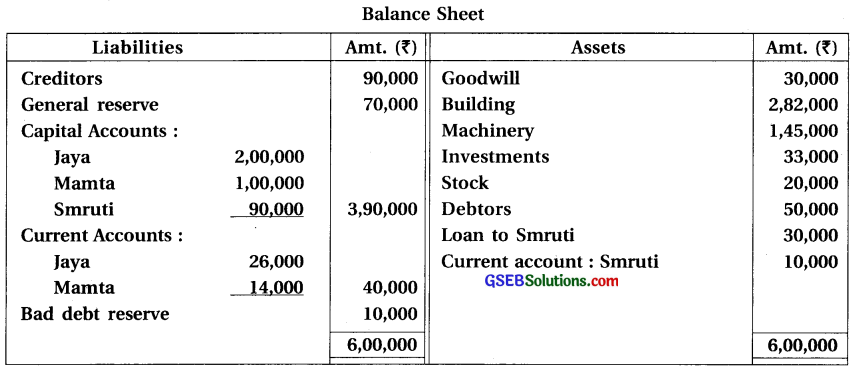Smruti retired on 1-4-2017 as a partner. At the time of her retirement, partners decided that:
(1) ₹ 4,000 is outstanding for rent payable. (2) Interest on investment is receivable ₹ 2,500. (3) Investments to be sold for ₹ 35,000. (4) Goodwill of the firm is valued at ₹ 2,00,000. (5) Jaya and Mamta will share future profit in the ratio of 1 : 1.
Prepare necessary accounts and balance sheet after retirement.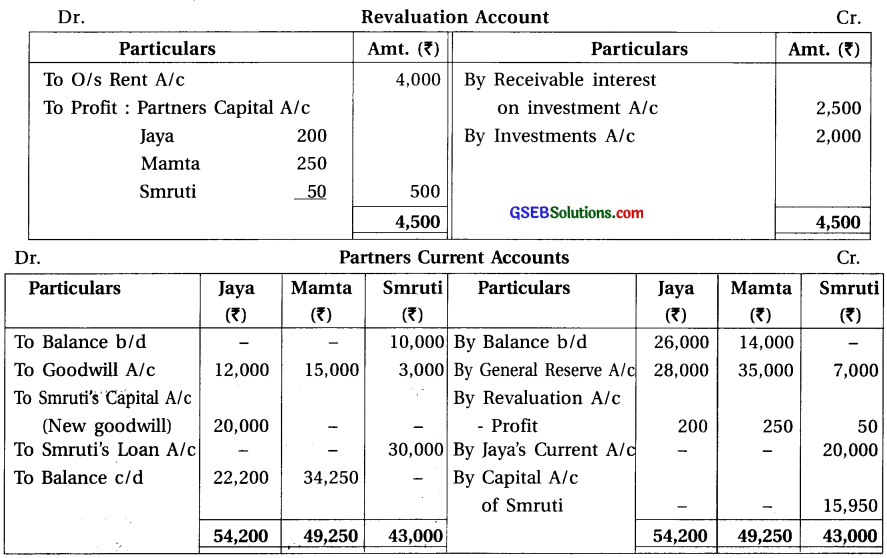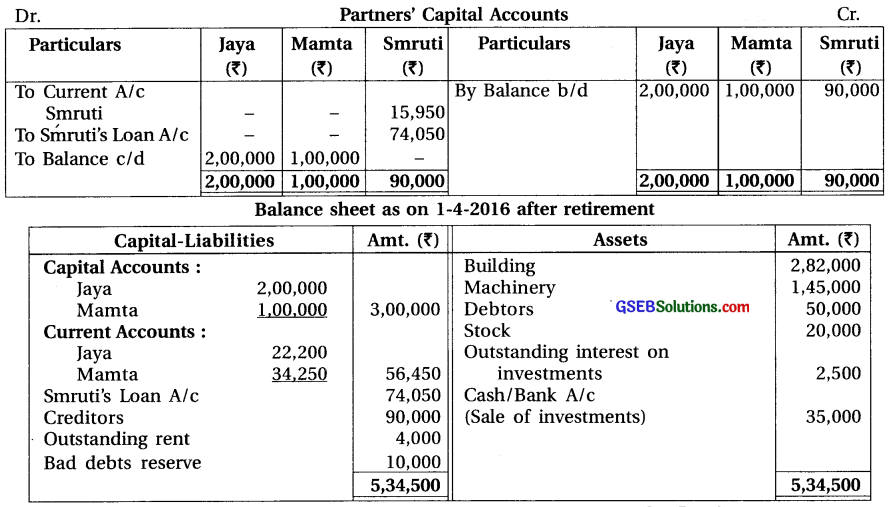Note :
(1) Gaining Ratio : Old ratio of Jaya, Mamta ans Smruti = $$\frac{2}{5}: \frac{5}{10}: \frac{1}{10}$$ = 4 : 5 : 1.
New ratio of Jaya and Mamta = 1 : 1.
Gain = New share – Old share
Gain of jaya = $$\frac{1}{2}-\frac{4}{10}=\frac{5-4}{10}=\frac{1}{10}$$
Gain of Mamta = $$\frac{1}{2}-\frac{5}{10}=\frac{5-5}{10}$$ = 0

(2) Share of goodwill of Smruti = ₹ 2,00,000 × $$\frac{1}{10}$$ = ₹ 20,000
Which is given by Jaya because gain of Mamta is zero. For this ₹ 20,000 will be debited to Jaya’s current A/c and credited to Smruti’s current A/c.

(3) Amount of old goodwill shown in the balance sheet ₹ 30,000 will be distributed between old partners in their old ratio (4 : 5 : 1).Question 9.
Madhav, Radha and Gopi are the partners sharing profit and loss in the ratio of $$\frac{1}{2}$$, $$\frac{1}{3}$$ and $$\frac{1}{6}$$. Balance sheet of the firm on 31-3-2016 was as under: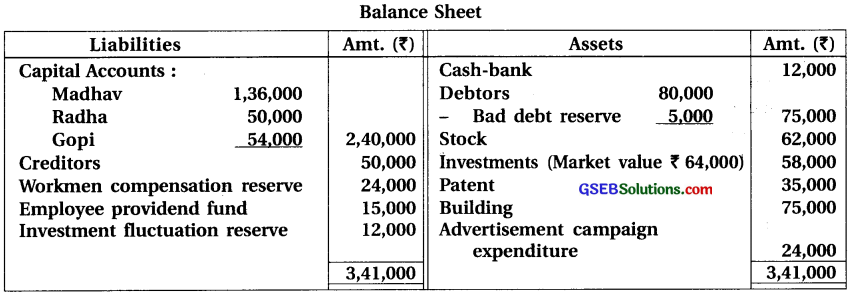Radha retired from 1-4-2016. Partners decided that:
(1) Value of patent is to be reduced by 20% whereas value of building is to be reduced to 90%. (2) Liability of workmen compensation reserve is decided at ₹ 30,000. (3) Bad debt reserve on debtors is to be increased by 5%. (4) ₹ 40,000 is to be paid to Radha as her share in goodwill. (5) ₹ 500 received from bad debt written off earlier ₹ 3,000. (6) ₹ 5,000 to be paid to Radha in cash and balance amount in two equal annual instalment with 10% interest per annum.
Prepare Revaluation account, Partners’ capital account and Balance sheet. Also prepare Radha’s loan account till it is finally paid.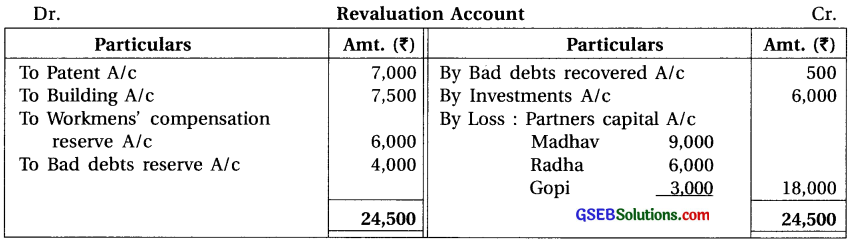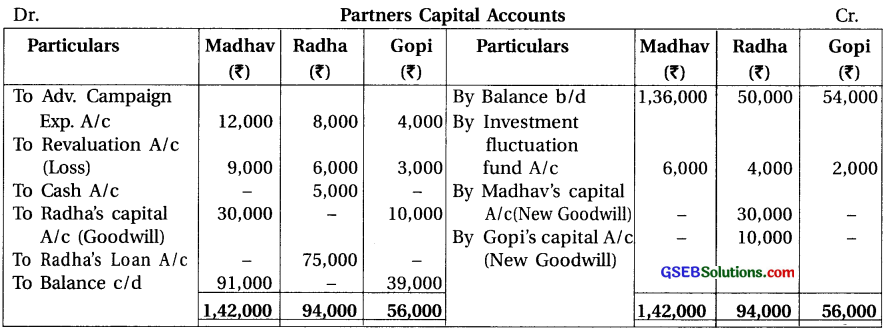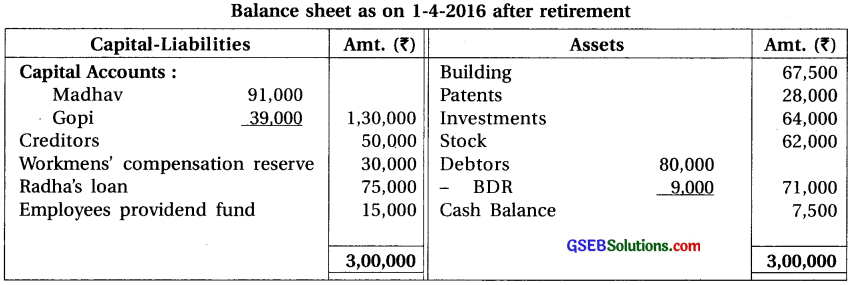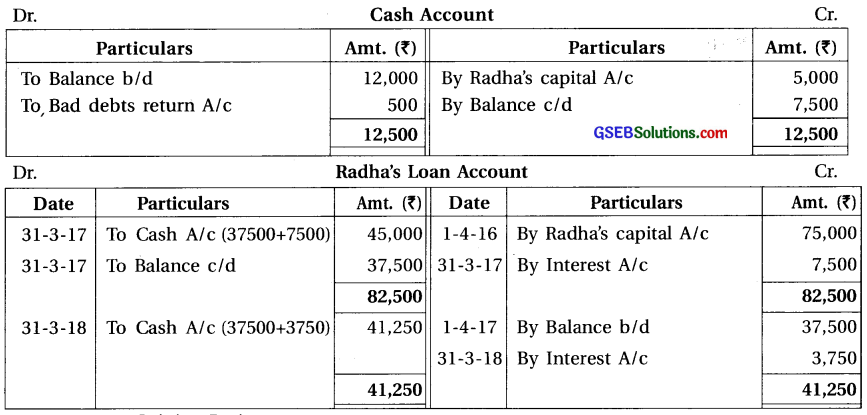Note :
(1) Gaining Ratio :
Old ratio of Madhav, Radha and Gopi = $$\frac{1}{2}: \frac{1}{3}: \frac{1}{6}$$ = 3 : 2 : 1.
Here, New ratio is not given after retirement of Radha, so new ratio of Madhav and Gopi = Old ratio = Gaining ratio = 3 : 1.

(2) Share of Radha’s goodwill = ₹ 40,000 which will be given to Madhav and Gopi in their gaining ratio (3 : 1).Question 10.
Deep, Jyoti and Geeta are the partners sharing profit and loss in the ratio of their capitals. Balance sheet of their firm as 31-3-2017 was as under :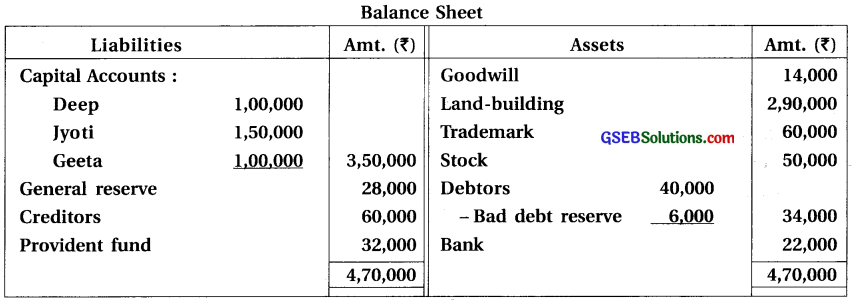Jyoti retired on the above date. Partners decided that,
(1) New profit and loss sharing ratio of Deep and Geeta is to be kept at 1:1. Goodwill of the firm valued at ₹ 70,000. (2) Bad debt reserve on debtors is to be reduced upto 10%. (3) Stock is shown in the books at 25% more than its cost, stock to be recorded at cost. (4) ₹ 60,000 is paid for trademark during current year, which is for total 6 years. (5) Liability of provident fund is decided at ₹ 35,000. (6) Total capital of the new firm is to be kept as the total capital of the old firm. Deep and Geeta will maintain this capital in their new profit-loss sharing ratio. For this purpose the difference is to be transferred to their current accounts.
Prepare necessary accounts and balance sheet after retirement.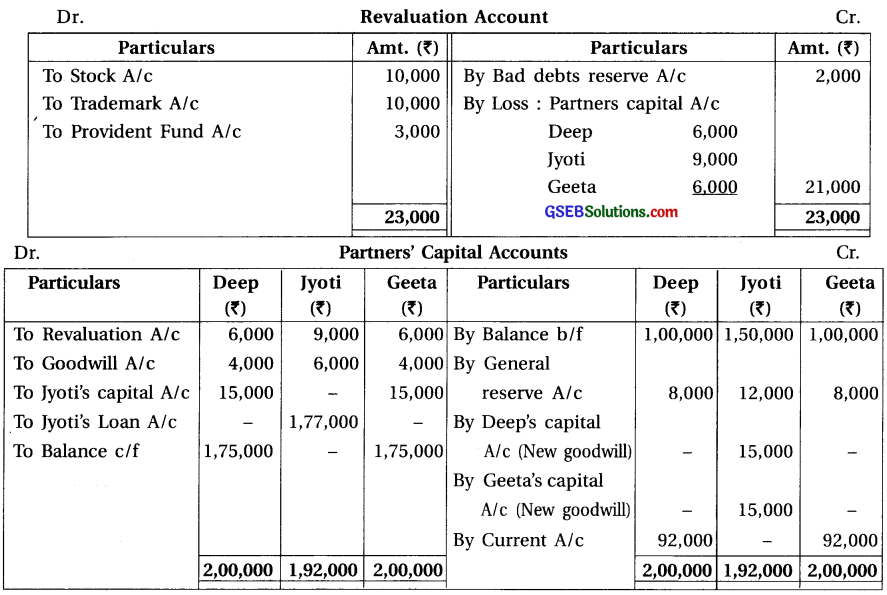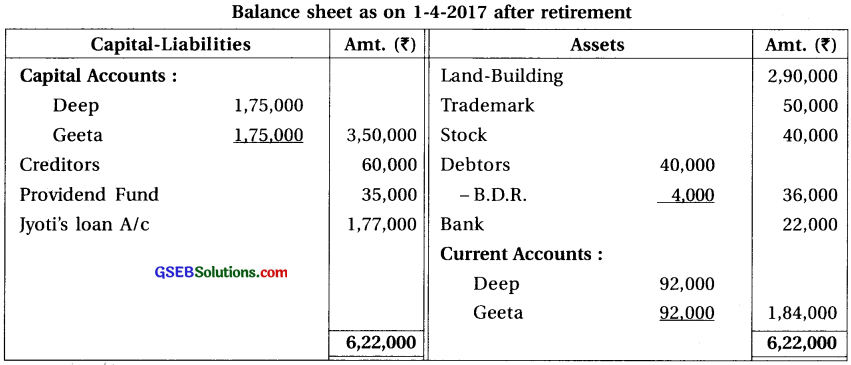Note : (1) Gaining Ratio :
Old ratio of Deep, Jyoti and Geeta (capital ratio) = 1,00,000 : 1,50,000 : 1,00,000 = 2 : 3 : 2. New ratio of Deep and Jyoti = 2 : 2 = 1 : 1.
Gain = New share – Old share.
Gain of Deep = $$\frac{1}{2}-\frac{2}{7}=\frac{7-4}{14}=\frac{3}{14}$$
Gain of Geetha = $$\frac{1}{2}-\frac{2}{7}=\frac{7-4}{14}=\frac{3}{14}$$
Gaining ratio of Deep and Jyoti = 3 : 3 = 1 : 1

(2) Share of Jyothi’s good will = ₹ 70,000 × $$\frac{3}{7}$$ = ₹ 30,000 Which will be given to Deep and Geeta in their gain ratio (1 : 1).

(3) Amount of old goodwill shown in the balance sheet will be distributed between old partners in their old ratio (2 : 3 : 2).

(4) Stock is shown in books at 25% more than its cost.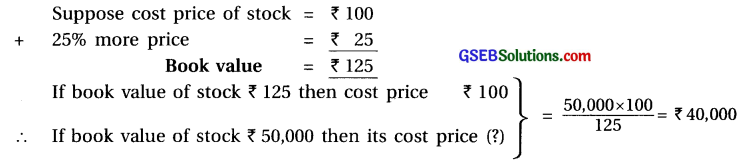So, the difference amount of stock ₹ 10,000 will be debited to Revaluation account.Question 11.
Moon, Star and Sun are the partners of a firm. Sun retires on 31-3-2017. Moon and Star will distribute future profit and loss in the ratio of 5 : 1. Balance sheet of their firm on 31-3-2017 was as under :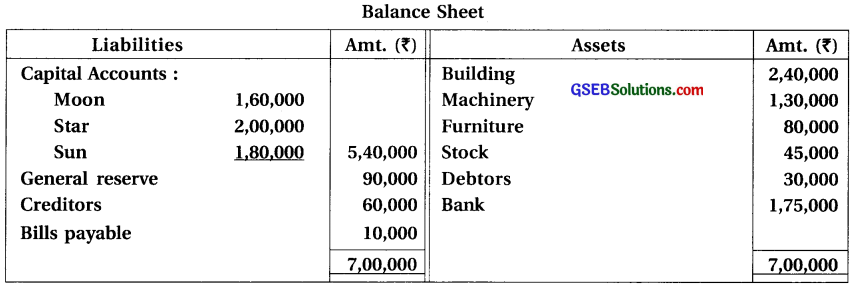Conditions of retirement were as under :
(1) Goodwill of the firm is valued at ₹ 60,000. (2) Creditors are payable after one month, which are to be paid immediately at 12% discount per annum. (3) Computer, written off from the books is now valued at ₹ 12,000. Moon will take over the computer at this value. (4) After retirement of Sun, Moon and Star will maintain their capital in the new profit and loss sharing ratio and difference is to be tansferred to bank account.
Prepare necessary accounts and balance sheet after retirement.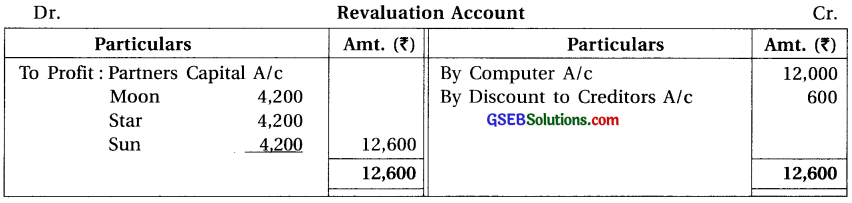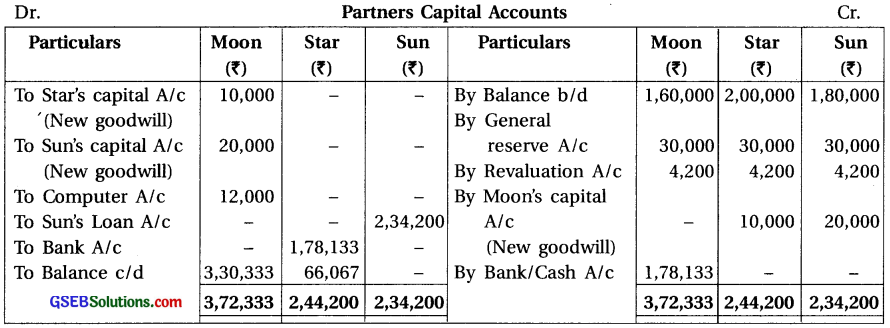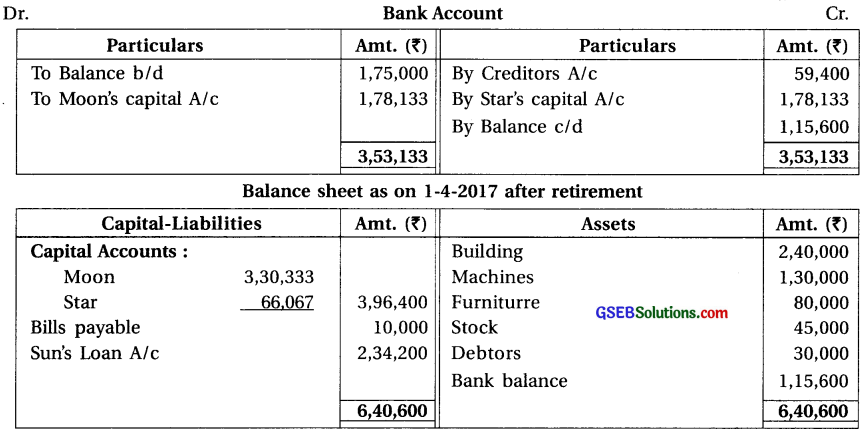Note : (1) Gaining Ratio :
Old ratio of Moon, Star and Sun = 1 : 1 : 1.
New ratio of Moon and Star = 5 : 1
Gain = New share – Old share.
∴ Gain of Moon = $$\frac{5}{6}-\frac{1}{3}=\frac{5-2}{6}=\frac{3}{6}$$
∴ Gain of star = $$\frac{1}{6}-\frac{1}{3}=\frac{1-2}{6}=\frac{-1}{6}$$ (Sacrifice)
∴ Only Moon will get the gain.

(2) Goodwill :
Goodwill received by Sun = ₹ 60,000 × $$\frac{1}{3}$$ = ₹ 20,000
Goodwill received by Star (Sacrifice) = ₹ 60,000 × $$\frac{1}{6}$$ = ₹ 10,000
Total amount of goodwill received by Moon (gain) = ₹ 20,000 + ₹ 10,000 = ₹ 30,000 which will be debited to his capital account and credited to Sun and Star’s capital account ₹ 20,000 and ₹ 10,000 respectively.

(3) Discount received on payment of creditors = ₹ 60,000 × $$\frac{12}{100}$$ × $$\frac{1}{12}$$ = ₹ 600

(4) Computer written off from the book at ₹ 12,000 will be profit so it will be credited to revaluation account.

(5) Capital of Moon and Star in New firm will be kept in new profit-loss sharing ratio (5 : 1).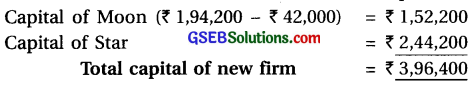New Capital of Moon = ₹ 3,96,400 × $$\frac{5}{6}$$ = ₹ 3,30,333
New Capital of Star = ₹ 3,96,400 × $$\frac{1}{6}$$ = ₹ 66,067
Here, Moon will bring ₹ 1,78,133 and Star will withdrawn ₹ 1,78,133.Question 12.
E, M and I are partners sharing profit and loss in the ratio of 5 : 3 : 2. Balance sheet of their firm on 31-3-2017 was as under :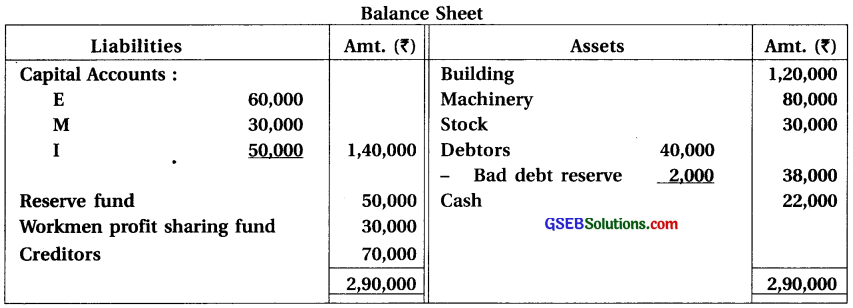I retired on 31-3-2017. Conditions of retirement were as under :
(1) I’s profit share will be gained by E and M in the ratio of 2 : 3. (2) Goodwill of the firm is valued at ₹ 1,00,000. (3) Bad debt reserve on debtors is to be increased by 10%. (4) Building is valued at 110%. (5) Value of machinery is to be reduced by 10 %. (6) Annual insurance premium of ₹ 24,000 is paid for the year ended on 30-6-2017. (7) E and M will maintain total capital of the firm ₹ 1,00,000 in their new profit and loss sharing ratio after retirement of I. Prepare necessary accounts and balance sheet after retirement.
Prepare necessary accounts and balance sheet after retirement.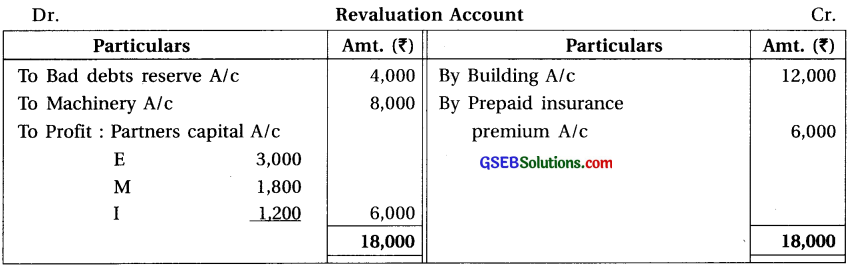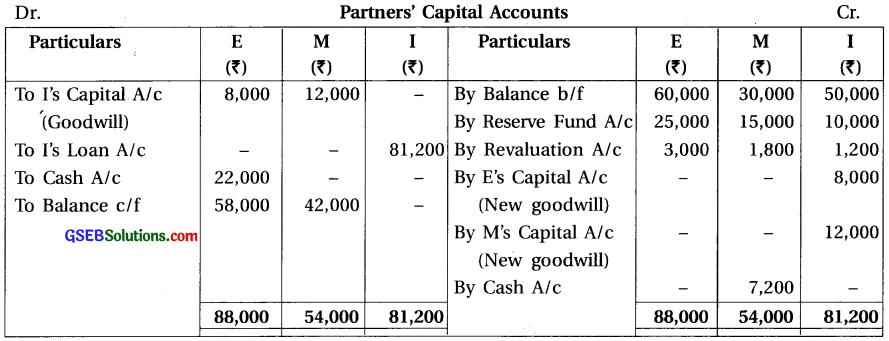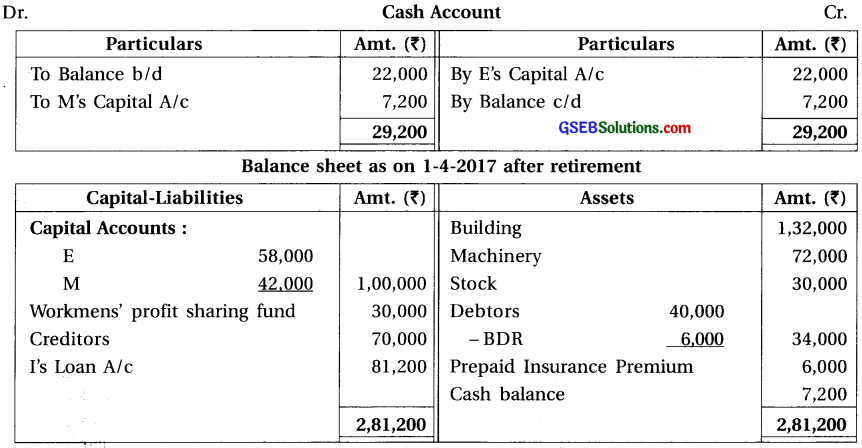Note :
(1) Gaining Ratio :
Old ratio of E, M and I = 5 : 3 : 2.
After retirement of I, his share will be obtained by E and M in the ratio of = 2 : 3
Gain of E = $$\frac{5}{6}-\frac{1}{3}=\frac{5-2}{6}=\frac{3}{6}$$ ∴ Gain of M = $$\frac{1}{6}-\frac{1}{3}=\frac{1-2}{6}=\frac{-1}{6}$$
Gaining ratio of E and M = 4 : 6 = 2 : 3
New Share = Old share + Gain
∴ New Share of E = $$\frac{5}{10}+\frac{4}{50}=\frac{25+4}{50}=\frac{29}{50}$$
∴ New Share of M = $$\frac{3}{10}+\frac{6}{50}=\frac{15+6}{50}=\frac{21}{50}$$
∴ New profit – loss sharing ratio of E and M = 29 : 21.

(2) Share of goodwill of I ₹ 1,00,000 × $$\frac{2}{10}$$ ₹ 20,000 which will be given to E and M in their gaining ratio (2 : 3).

(3) Total capital of New firm ₹ 1,00,000 which will be kept in new profit-loss sharing ratio of E and M (29 : 21).
New capital of E = ₹ 1,00,000 × $$\frac{29}{50}$$ = ₹ 58,000
New capital of M = ₹ 1,00,000 × $$\frac{21}{50}$$ = ₹ 42,000
For this E will withdrawn ₹ 22,000 cash and M will bring ₹ 7,200 cash.Question 13.
L, B and W are the partners of a firm sharing profit and loss in the ratio of 2 : 2 : 1. Balance sheet of their firm on 31-3-2016 was as under :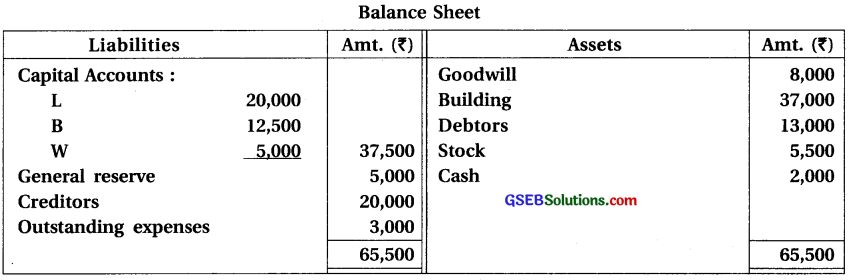W retired on 1-4-2016. Terms of retirement were decided as under :
(1) Market value of building is ₹ 50,000. (2) Book value of stock is 10% more than its cost. Stock is to be recorded at its cost. (3) Personal expenses of W ₹ 500 was debited to profit and loss
account. (4) Goodwill of the firm is valued at ₹ 80,000. (5) L will gain $$\frac {5}{40}$$ and B will gain $$\frac {3}{40}$$ from W’s share of profit. (6) Amount due to W is to be paid in cash and the same amount will be brought in cash by L and B in such a manner that their capital may remain in their new profit-loss sharing ratio in the new firm. Prepare necessary accounts and balance sheet of the new firm.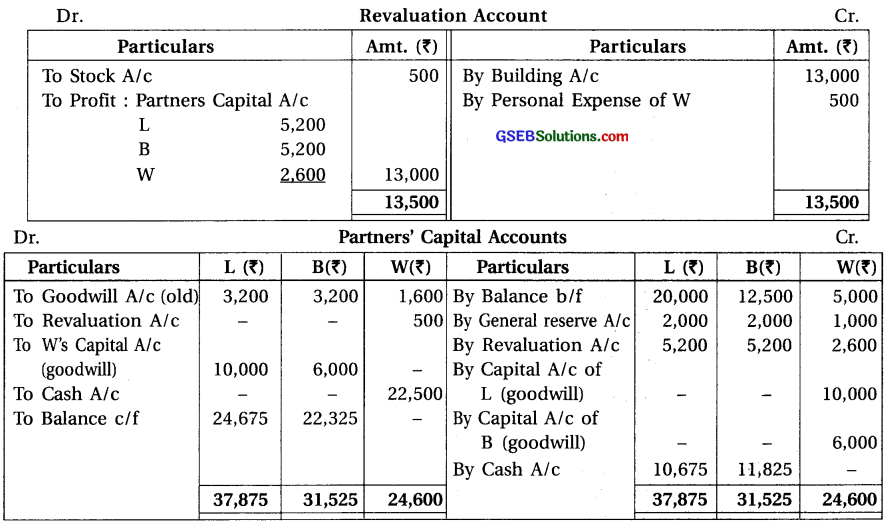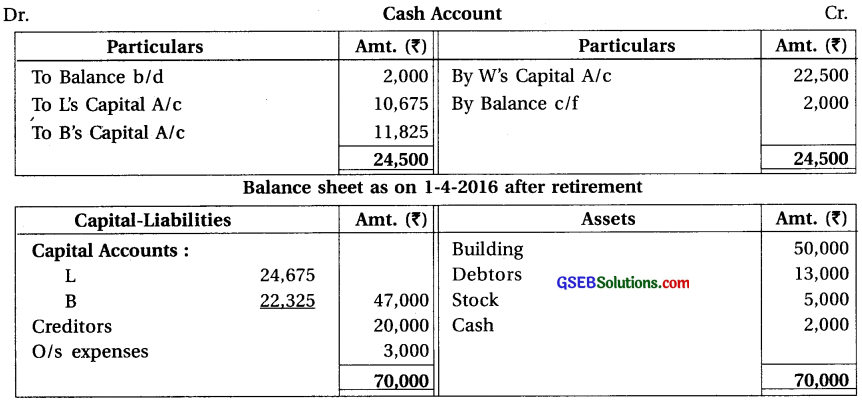Note :
(1) New Profit and Loss Ratio :
Old ratio of E, M and I = 2 : 2 : 1.
From the profit share of retired partner W, $$\frac {5}{40}$$ share received by L and $$\frac {3}{40}$$ share received by B. ∴ Gaining Ratio = $$\frac {5}{40}$$ : $$\frac {3}{40}$$ , 5 : 3.
New share = Old share + Gain
∴ New share of L = $$\frac{2}{5}+\frac{5}{40}=\frac{16+5}{40}=\frac{21}{40}$$
∴ New share of B = $$\frac{2}{5}+\frac{3}{40}=\frac{16+3}{40}=\frac{19}{40}$$
∴ New ratio of L and B = 21 : 19

(2) Share of goodwill of W = ₹ 80,000 × $$\frac{1}{5}$$ ₹ 16,000; which will be given to L and B in their gaining ratio (5 : 3).

(3) Amount of old goodwill shown in the balance sheet ₹ 8,000 will be distributed between old partners in their old ratio (2 : 2 : 1).

(4) Amount paid to retired partner W ₹ 22,000 and calcultion of new capital of L and B:
(i) Amount paid to W ₹ 22,500 will be debited to his capital account and credited to cash account.
(ii) In new balance sheet of the firm, show the new value of all assets and closing cash balance ₹ 2,000 after revaluation. Put the total of assets side ₹ 70,000 and show the same amount on Liability side ₹ 70,000.
(iii) On liability side of balance sheet; creditors ₹ 20,000 and unpaid expenses ₹ 3,000. So total amount of liability ₹ 20,000 will be deducted from ₹ 70,000. So difference amount ₹ 47,000 will be the capital of L and B. This amount will be distributed between them in their-new profit and loss sharing ratio (21 : 19).
New capital of L = ₹ 47,000 × $$\frac{21}{40}$$ = ₹ 24,675 and
New capital of B = ₹ 47,000 × $$\frac{19}{40}$$ = ₹ 22,325

(5) This capital will be shown on debit side on their capital account respectively and the difference amount of capital account on credit side ₹ 10,675 and ₹ 11,825 will be debited to cash account.Question 14.
Chirag, Jigar and Keshav are the partners sharing profit and loss in ratio of 3 : 2 : 1. Balance sheet of their firm on 31-3-2016 was as under :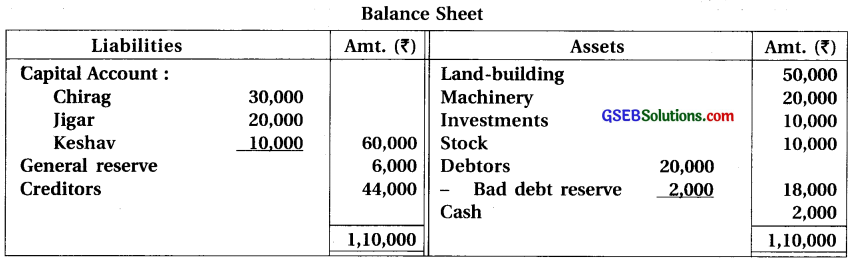Keshav retired on 31-3-2016. Following conditions were decided at the time of retirement :
(1) Value of land-building is to be increased by 20%. (2) Machinery is valued at 90% of its book value. (3) Market value of investment is 150% of its book value. (4) Bad debt reserve on debtors is to be reduced by 5%. (5) Goodwill of the firm is valued at ₹ 36,000. (6) ₹ 2,000 is outstanding for salary payable to an employee. (7) Chirag and Jigar will bring necessary amount in cash in such a manner that amount due to Keshav is to be paid in cash and balance of cash may remain in the firm as working capital ₹ 14,000 and their capital in the new firm become proportionate to their new profit-loss sharing ratio.
Prepare necessary accounts and balance sheet of new firm.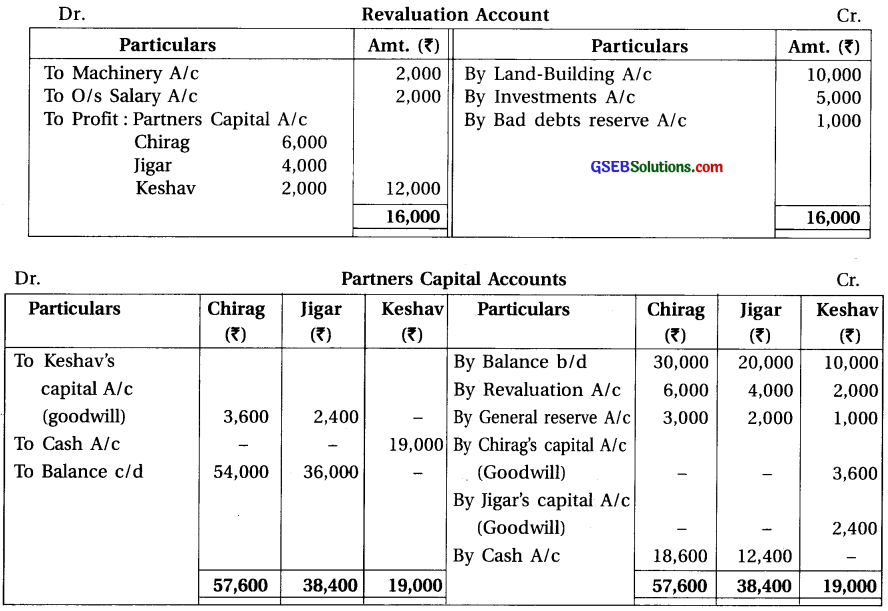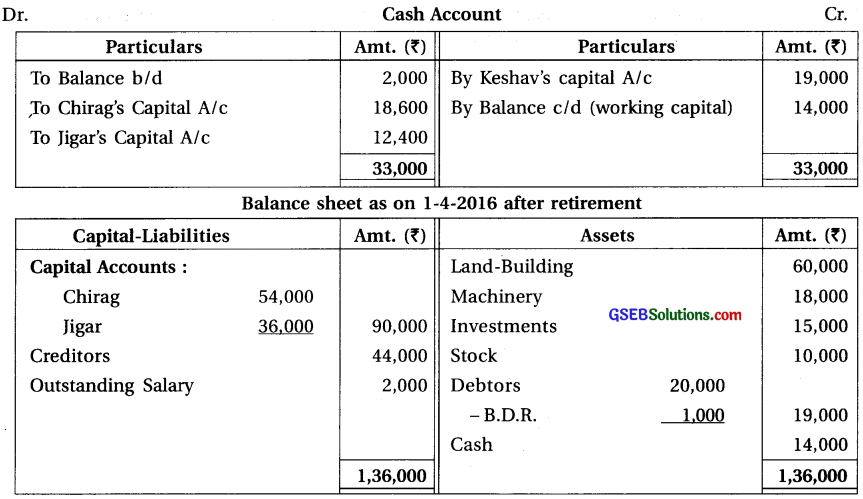Note : (1) Gaining Ratio and New Profit and Loss Ratio :
Old ratio of Chirag, Jigar and Keshav = 3 : 2 : 1.
There is no information regarding new ratio after retirement of Keshav. New ratio of Chirag and Jigar = Old ratio = Gaining ratio 3 : 2.

(2) Share of goodwill of Keshav = ₹ 36,000 × $$\frac{1}{6}$$ = ₹ 6,000; which will be given to Chirag and Jigar in their gaining ratio (3 : 2).

(3) Calculation of new capital of Chirag and Jigar in new firm :
(i) Amount paid to Keshav ₹ 19,000 will be debited to his capital account and credited to cash account.
(ii) Write closing balance as balance c/d on credit side of cash account ₹ 14,000 and on asset side of new balance sheet. Show this cash balance ₹ 14,000 and put total of assets side ₹ 1,36,000 and put same total on liability side ₹ 1,36,000.
(iii) On liability side, deducting ₹ 46,000 (creditors ₹ 44,000 + unpaid salary ₹ 2,000) from ₹ 1,36,000, difference amount ₹ 90,000 which will be the capital of Chirag and Jigar. This will be distributed in new profit-loss sharing ratio (3 : 2).
New capital of Chirag = ₹ 90,000 × $$\frac{3}{5}$$ = ₹ 54,000 and
New capital of Jigar = ₹ 90,000 × $$\frac{2}{5}$$ = ₹ 36,000.
(iv) Show this balance of capital on debit side of capital account of Chirag and Jigar. The difference amount on credit side of their capital account ₹ 18,600 and ₹ 12,400 will be debited to cash account.Question 15.
E, F and G are the partners sharing profit and loss in the ratio of 4 : 3 : 3. E retires on 31-3-2017. Balance sheet of the firm on that date was as under :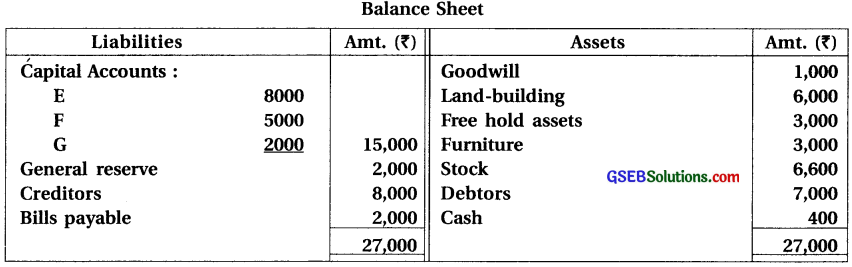Following terms of retirement were decided in partnership agreement and among the partners:
(1) Goodtvill of the firm is valued at ₹ 7,000. (2) Value of land-building ₹ 7,000 and furniture ₹ 2,000 is decided. (3) Stock is over valued by 10%. ₹ 5000 to be paid to E immediately and balance to be transferred to his loan account. (4) F and G will bring necessary cash in equal proportion in such a manner that E is to be paid his dues fully and ₹ 2,000 remain as working capital (cash).
Prepare profit and loss adjustment account, cash account, partners’ capital accounts and balance sheet.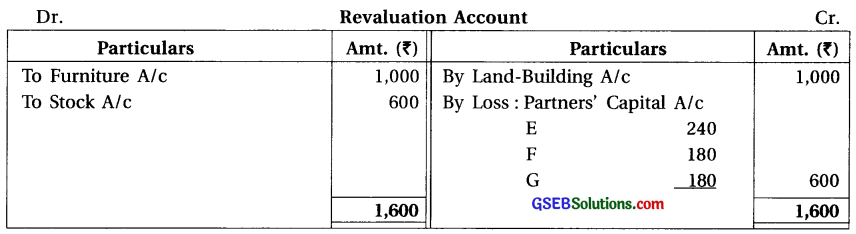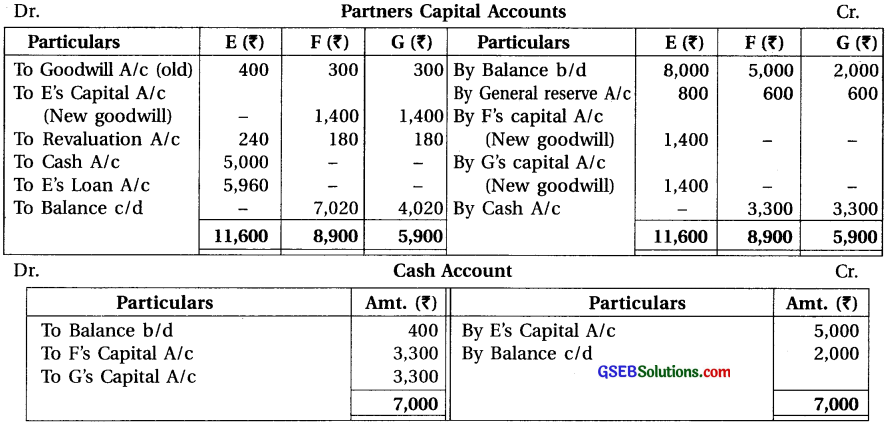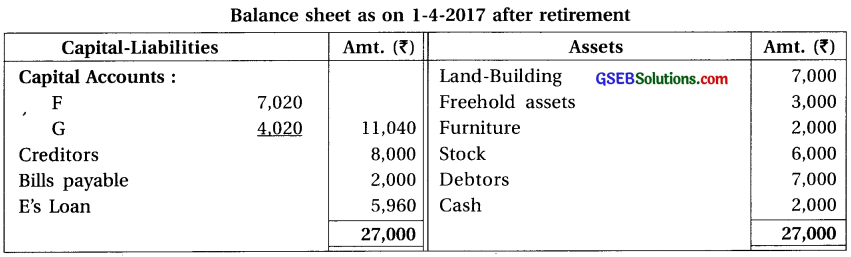Note : (1) New Profit and Loss Ratio and Gaining Ratio :
Old ratio of E, F and G = 4 : 3 : 3.
There is no information regarding new ratio after retirement of E.
New ratio of F and G = Old ratio = Gaining ratio 3 : 3 = 1 : 1.

(2) Share of goodwill of E = ₹ 7,000 × $$\frac {4}{10}$$ = ₹ 2,800; which will be given to F and G in their gaining ratio (1 : 1).

(3) Amount of old goodwill shown in the balance sheet will be written off between old partners in their old ratio (4 : 3 : 3)

(4) Amount paid to retiring partner E ₹ 5,000 and ₹ 2,000 kept as a working capital so total amount ₹ 7,000, from which opening cash balance ₹ 400 will be deducted. So difference amount ₹ 6,600 which will be bring by F and G in equal ratio (₹ 3,300 each).Question 16.
Kamal, Bimal and Vimal are the partners sharing profit and loss in the ratio of 1:2:3. Their balance sheet shows following balances on 31-3-2016.
Capital : Kamal ₹ 10,000; Bimal ₹ 20,000 and Vimal ₹ 30,000
General reserve ₹ 24,000; Loan of Vimal ₹ 10,000; Goodwill ₹ 12,000
Vimal died on 31-5-2016. Following conditions were provided in partnership deed :
(1) Goodwill of the firm is to be valued at two years purchase of average profit of last three years. Profit of last three years were ₹ 25,000; ₹ 40,000 and ₹ 25,000. (2) Profit share of the deceased partner till the date of death is to be decided on the basis of average profit of last three years. (3) Interest on capital is to be allowed at 12% per annum. Prepare Vimal’s capital account.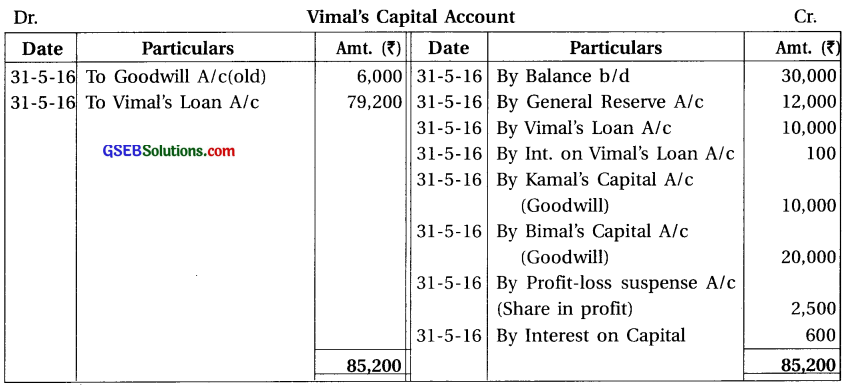Note :
(1) Goodwill : Old = 12,000 × $$\frac {3}{6}$$ = ₹ 6,000

(2) Goodwill : New = Average profit of 3 years = $$\frac{90,000}{3}$$= ₹ 30,000
„ Here goodwill is to be calculated of average profit of 3 years of 2 years purchase,
i.e. 30,000 × 2 = ₹ 60,000. Vimal’s share in goodwill = 60,000 × $$\frac {3}{6}$$ = ₹ 30,000.

(3) Vimal’s share in profit is to be calcualted based on last three year’s average profit, to the date of death, i.e. 2 month’s profit is to be calculated = 30,000 (Avg. Profit)
× $$\frac{2}{12} \times \frac{3}{6}$$ = ₹ 2’500

(4) Interest on capital @ 12% = 30,000 × $$\frac{12}{100} \times \frac{2}{12}$$ = ₹ 600.

(5) Interest on loan = 10,000 × $$\frac{6}{100} \times \frac{2}{12}$$ = ₹ 100

Question 17.
C, S and T are the partners of a firm sharing profit and loss in the ratio of 2 : 1 : 2. Balance sheet of their firm on 31-3-2017 was as under :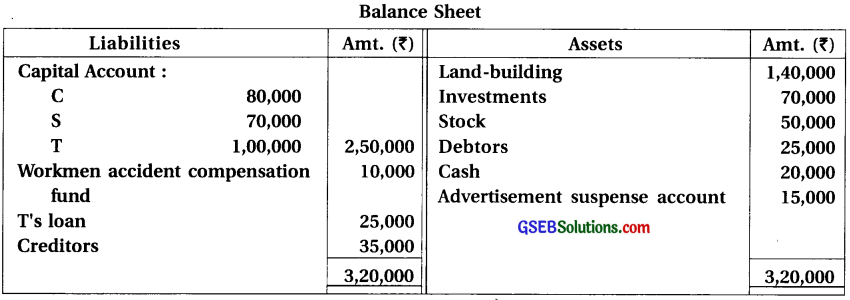T died on 30-6-2017. As per partnership deed, following accounting treatments are necessary to be given at the time of death of a partner :
(1) Interest on capital is to be allowed at 6% per annum. (2) Interest on drawings is to be charged at 12% p.a. T withdrew ₹ 20,000 on 1-5-2017. (3) Goodwill is to be valued at two times the average profit of last three years : Profit of last three years was as under :
2014-15 ₹ 80,000; 2015-16 ₹ 75,000 and 2016-17 ₹ 85,000
(4) Profit share to be given on the basis of profit of previous year.
Prepare T’s executor’s account.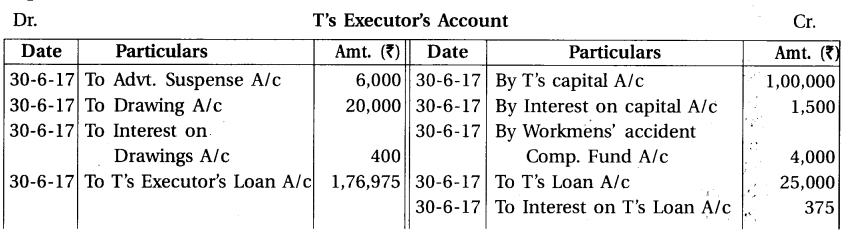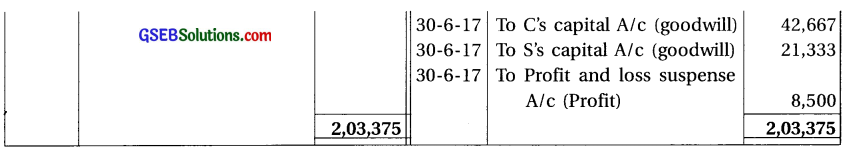Note :
(1) Capital of T ₹ 1,00,000 which will be credited to T’s executor’s account.
Interest on capital of T = 1,00,000 × $$\frac{6}{100} \times \frac{3}{12}$$ = ₹ 1,500

(2) Loan of T ₹ 25,000 which will be credited of T’s excutors account.
Interest on loan of T = 25,000 × $$\frac{6}{100} \times \frac{3}{12}$$ = ₹ 375

(3) Share of T in workmens’ accident compensation fund = 10,000 × $$\frac{2}{5}$$ = ₹ 4,000.

(4) Share of T in Advt. Suspense account = ₹ 15,000 × $$\frac{2}{5}$$ = ₹ 6,000 which will be debited to T’s executor account.

(5) New Goodwill : Average Profit = $$\frac{80,000+75,000+85,000}{3}$$ = ₹ 80,000
Goodwill of the firm = ₹ 80,000 × 2 = ₹ 1,60,000
∴ Share of T in Goodwill = ₹ 1,60,000 × $$\frac{2}{5}$$ ₹ 64,000 which will be given to C and S in their gaining ratio (2 : 1).

(6) Profit share of T till death :
Date of Death of T is 30-6-17
∴ From the Dt.1-4-17 to Dt. 30-6-17, means 3 month profit will be calculated.
∴ Profit share of T = ₹ 85,000 × $$\frac{3}{12} \times \frac{2}{5}$$ = ₹ 8,500Question 18.
E, V and M are the partners of a firm sharing profit and loss in the ratio of 3 : 2 : 1. Balance sheet of their firm on 31-3-2016 was as under :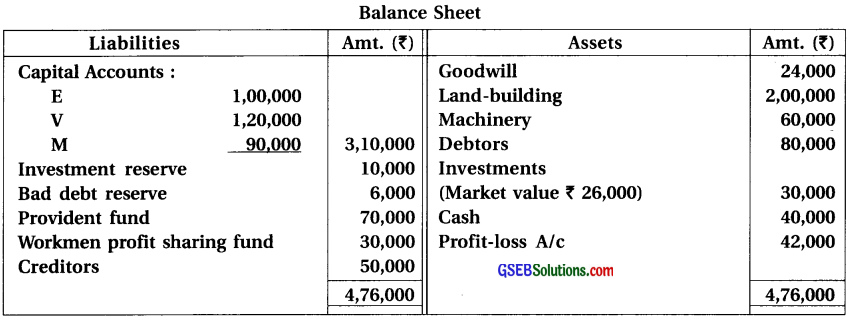V died on 1-10-2016. As per partnership deed, following was decided among partners :
(1) Goodwill is valued at ₹ 1,20,000. (2) New profit-loss sharing ratio of E and M decided at 2 : 1. (3) V is to be given profit share till the date of his death on the basis of the profit of last year.
(4) 10% reserve for bad debt to be provided on debtors. (5) Land and building is valued 10% more.(6) ₹ 1,000 salary per month is payable to V. (7) ₹ 45,000 to be paid to V’s executor immediately and balance amount in two equal yearly instalments with interest at 10% per annum.
Prepare Vs executor account and executor’s loan account till it is finally paid.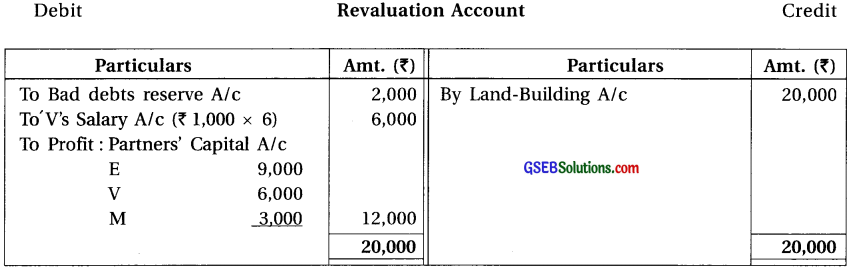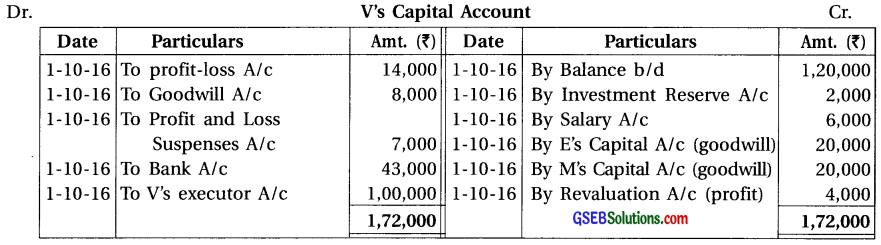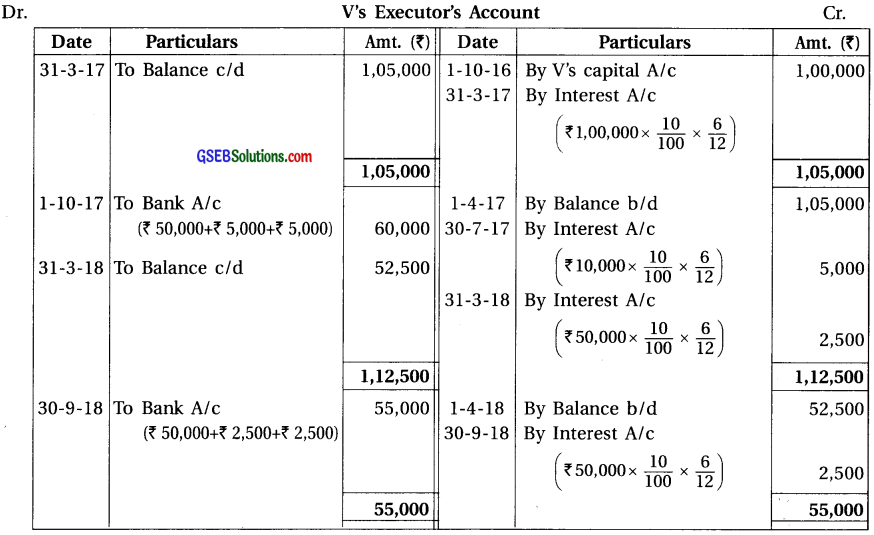Note :
(1) Book value of Investment is ₹ 30,000 and its market value is ₹ 26,000. So the difference
amount ₹ 4,000 will be deducted from investment reserve, so remaining amount ₹ 6,000 will be distributed between partners. In which the share of died partner V ₹ 6,000 × $$\frac {2}{6}$$ = ₹ 2,000

(2) Debit balance of profit and loss account in the balance sheet ₹ 42,000 in which share of V = ₹ 42,000 × $$\frac {2}{6}$$ = ₹ 14,000 which will be debited to its capital account.

(3) Old goodwill shown in the balance sheet of the firm ₹ 24,000 will be written off. In which share of V = ₹ 24,000 × $$\frac {2}{6}$$ = ₹ 8,000 which willbe debited to its capital account.

(4) Gaining Ratio :
Old ratio of E, V and M = 3 : 2 : 1.
New ratio of E and M = 2 : 1.
Gain = New sahre – Old share
∴ Gain of E = $$\frac{2}{3}-\frac{3}{6}=\frac{4-3}{6}=\frac{1}{6}$$
∴ Gain of M = $$\frac{1}{3}-\frac{1}{6}=\frac{2-1}{6}=\frac{1}{6}$$
∴ Gaining ratio of E and M = 1 : 1.

(5) Share of Goodwill of V = ₹ 1,20,000 × $$\frac {2}{6}$$ = ₹ 40,000 which will be given to E and M in their gaining ratio (1 : 1).

(6) Profit share of V till his death :
Date of death of V is 1-10-16
∴ He will receive profit from 1-4-16 to 1-10-16 (six month)
∴ Last year it was loss ₹ 42,000
Share of V in loss = ₹ 42,000 × $$\frac {6}{12}$$ × $$\frac {2}{6}$$ = ₹ 7,000 which will be debited to his capital account.Question 19.
A, T and M are the partners sharing profit and loss in the ratio of 4 : 1 : 1. Balance sheet of their firm on 31-3-2017 was as under :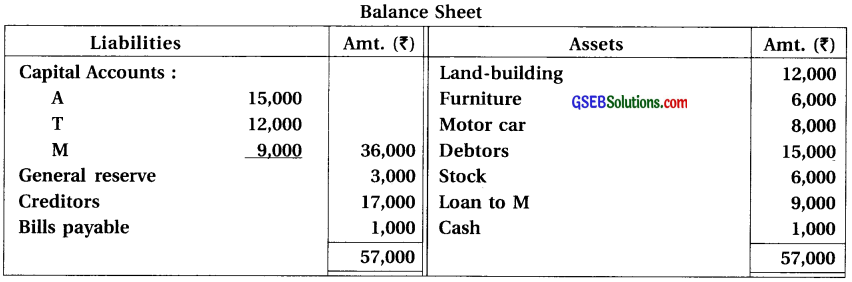M died on 1-7-2017. As per partnership agreement among partners :
(1) Value of land and building and furniture is to be increased by 10%. (2) Cost of stock is ₹ 5,500, which is to be brought in the books. (3) ₹ 400 to be written off as bad debt from debtors.
(4) Goodwill is valued at ₹ 7,200. (5) M is to be given share in profit till the date of his death on basis of sales and net profit of last year. Sales of last year was ₹ 8,00,000. Sales of first three months of Current year was ₹ 4,00,000. Net profit of last year was ₹ 2,40,000. (6) ₹ 1,850 to be paid to NTs executor in cash and balance amount in two equal annual instalments with interest at 12% interest per annum.
Prepare M’s executor’s account and executor’s loan account till it is finally paid.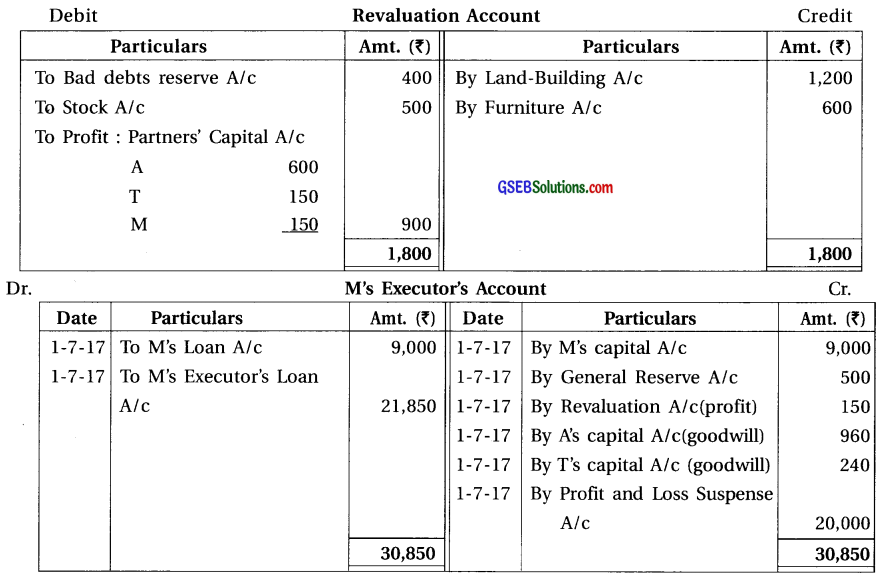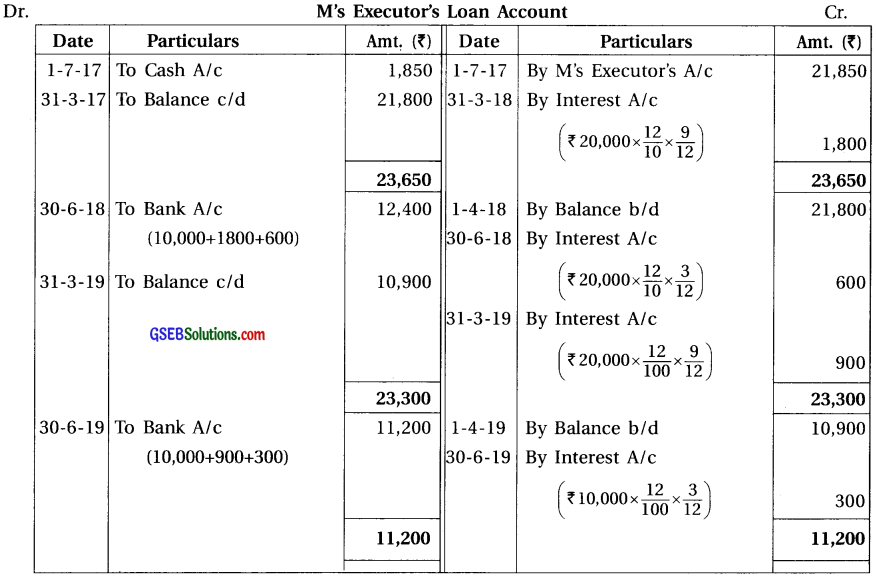(1) Share of M in general reserve = ₹ 3,000 × $$\frac {1}{6}$$ = ₹ 500
(2) Share of M in goodwill = ₹ 7,200 × $$\frac {1}{6}$$ = ₹ 1,200 which will be given to A and T in their gain ratio (old ratio) (4 : 1).
= Sale ×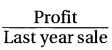× M share in profit
= ₹ 4,00,000 $$\frac{2,40,000}{8,00,000}$$ × $$\frac {1}{6}$$ = ₹ 20,000